• 单变量时间序列预测 数据类型：单列 ​ import numpy import matplotlib.pyplot as plt from keras.models import Sequential from keras.layers import Dense from keras.layers import LSTM from keras....
单变量时间序列预测

数据类型：单列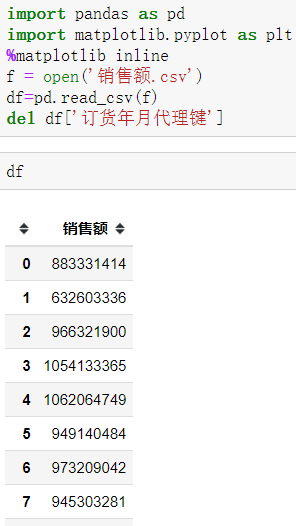​
import numpy
import matplotlib.pyplot as plt
from keras.models import Sequential
from keras.layers import Dense
from keras.layers import LSTM

dataset = df.values
# 将整型变为float
dataset = dataset.astype('float32')

from sklearn.preprocessing import MinMaxScaler
scaler = MinMaxScaler(feature_range=(0, 1))
dataset = scaler.fit_transform(dataset)
train_size = int(len(dataset) * 0.65)
trainlist = dataset[:train_size]
testlist = dataset[train_size:]

def create_dataset(dataset, look_back):
#这里的look_back与timestep相同
dataX, dataY = [], []
for i in range(len(dataset)-look_back-1):
a = dataset[i:(i+look_back)]
dataX.append(a)
dataY.append(dataset[i + look_back])
return numpy.array(dataX),numpy.array(dataY)
#训练数据太少 look_back并不能过大
look_back = 1
trainX,trainY  = create_dataset(trainlist,look_back)
testX,testY = create_dataset(testlist,look_back)
trainX = numpy.reshape(trainX, (trainX.shape, trainX.shape, 1))
testX = numpy.reshape(testX, (testX.shape, testX.shape ,1 ))
# create and fit the LSTM network
model = Sequential()
model.fit(trainX, trainY, epochs=100, batch_size=1, verbose=2)
# model.save(os.path.join("DATA","Test" + ".h5"))
# make predictions

#模型验证
trainPredict = model.predict(trainX)
testPredict = model.predict(testX)

#反归一化
trainPredict = scaler.inverse_transform(trainPredict)
trainY = scaler.inverse_transform(trainY)
testPredict = scaler.inverse_transform(testPredict)
testY = scaler.inverse_transform(testY)
plt.plot(trainY)
plt.plot(trainPredict[1:])
plt.show()s
plt.plot(testY)
plt.plot(testPredict[1:])
plt.show()

​

预测未来数据方法

.单维单步（使用前n（2，代码演示为3）步预测后一步）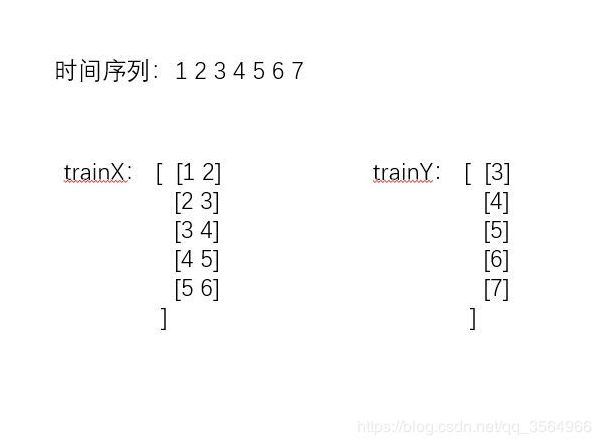#对全部数据进行训练
import numpy
import matplotlib.pyplot as plt
from keras.models import Sequential
from keras.layers import Dense
from keras.layers import LSTM

dataset = df.values
# 将整型变为float
dataset = dataset.astype('float32')
from sklearn.preprocessing import MinMaxScaler
scaler = MinMaxScaler(feature_range=(0, 1))
dataset = scaler.fit_transform(dataset)
def create_dataset(dataset, look_back):
#这里的look_back与timestep相同
dataX, dataY = [], []
for i in range(len(dataset)-look_back-1):
a = dataset[i:(i+look_back)]
dataX.append(a)
dataY.append(dataset[i + look_back])
return numpy.array(dataX),numpy.array(dataY)
#训练数据太少 look_back并不能过大
look_back = 1
trainX,trainY  = create_dataset(dataset,look_back)
trainX = numpy.reshape(trainX, (trainX.shape, trainX.shape, 1))
# create and fit the LSTM network
model = Sequential()
model.fit(trainX, trainY, epochs=100, batch_size=1, verbose=2)

######################滚动预测########
predict_xlist = []#添加预测x列表
predict_y = []#添加预测y列表
timesteps=1
length=12
predict_xlist.extend(dataset[dataset.shape-timesteps:dataset.shape,0].tolist())#已经存在的最后timesteps个数据添加进列表，预测新值(比如已经有的数据从1,2,3到288。现在要预测后面的数据，所以将216到288的72个数据添加到列表中，预测新的值即288以后的数据）
while len(predict_y) < length:
predictx = np.array(predict_xlist[-timesteps:])#从最新的predict_xlist取出timesteps个数据，预测新的predict_steps个数据（因为每次预测的y会添加到predict_xlist列表中，为了预测将来的值，所以每次构造的x要取这个列表中最后的timesteps个数据词啊性）
predictx = np.reshape(predictx,(1,timesteps,1))#变换格式，适应LSTM模型
#print("predictx"),print(predictx),print(predictx.shape)
#预测新值
lstm_predict = model.predict(predictx)
#predict_list.append(train_predict)#新值y添加进列表，做x
#滚动预测
#print("lstm_predict"),print(lstm_predict)
predict_xlist.extend(lstm_predict)#将新预测出来的predict_steps个数据，加入predict_xlist列表，用于下次预测
# invert
lstm_predict = scaler.inverse_transform(lstm_predict)
predict_y.extend(lstm_predict)#预测的结果y，每次预测的12个数据，添加进去，直到预测288个为止



单维多步（使用前两步预测后两步）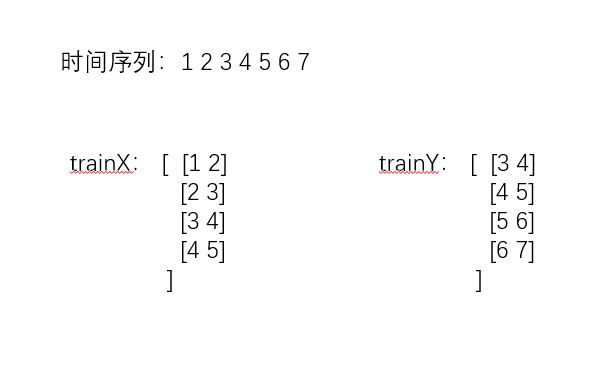from numpy import array

# split a univariate sequence into samples
def split_sequence(sequence, n_steps_in, n_steps_out):
X, y = list(), list()
for i in range(len(sequence)):
# find the end of this pattern
end_ix = i + n_steps_in
out_end_ix = end_ix + n_steps_out
# check if we are beyond the sequence
if out_end_ix > len(sequence):
break
# gather input and output parts of the pattern
seq_x, seq_y = sequence[i:end_ix], sequence[end_ix:out_end_ix]
X.append(seq_x)
y.append(seq_y)
return array(X), array(y)

raw_seq = [10, 20, 30, 40, 50, 60, 70, 80, 90]
# 也就是用之前的三步，预测之后的2步
n_steps_in, n_steps_out = 3, 2
X, y = split_sequence(raw_seq, n_steps_in, n_steps_out)
for i in range(len(X)):
print(X[i], y[i])

'''
[10 20 30] [40 50]
[20 30 40] [50 60]
[30 40 50] [60 70]
[40 50 60] [70 80]
[50 60 70] [80 90]
'''
# reshape from [samples, timesteps] into [samples, timesteps, features]
n_features = 1
X = X.reshape((X.shape, X.shape, n_features))
# define model
model = Sequential()
# 和之前单个步不同点在于 这一层的神经元=n_steps_out

model.fit(X, y, epochs=50, verbose=0)
# demonstrate prediction
x_input = array([70, 80, 90])
x_input = x_input.reshape((1, n_steps_in, n_features))
yhat = model.predict(x_input, verbose=0)
print(yhat)                                      # [[107.168686 118.79841 ]]


多变量时间序列预测

#######################################多变量，多步骤预测
from numpy import array
from numpy import hstack
from keras.models import Sequential
from keras.layers import LSTM
from keras.layers import Dense

# split a multivariate sequence into samples
def split_sequences(sequences, n_steps_in, n_steps_out):
X, y = list(), list()
for i in range(len(sequences)):
# find the end of this pattern
end_ix = i + n_steps_in
out_end_ix = end_ix + n_steps_out-1
# check if we are beyond the dataset
if out_end_ix > len(sequences):
break
# gather input and output parts of the pattern
seq_x, seq_y = sequences[i:end_ix, :-1], sequences[end_ix-1:out_end_ix, -1]
X.append(seq_x)
y.append(seq_y)
return array(X), array(y)

# define input sequence
in_seq1 = array([10, 20, 30, 40, 50, 60, 70, 80, 90])
in_seq2 = array([15, 25, 35, 45, 55, 65, 75, 85, 95])
out_seq = array([in_seq1[i]+in_seq2[i] for i in range(len(in_seq1))])
# convert to [rows, columns] structure
in_seq1 = in_seq1.reshape((len(in_seq1), 1))
in_seq2 = in_seq2.reshape((len(in_seq2), 1))
out_seq = out_seq.reshape((len(out_seq), 1))
# horizontally stack columns
dataset = hstack((in_seq1, in_seq2, out_seq))
# choose a number of time steps
n_steps_in, n_steps_out = 3, 2
# covert into input/output
X, y = split_sequences(dataset, n_steps_in, n_steps_out)
# the dataset knows the number of features, e.g. 2
n_features = X.shape
# define model
model = Sequential()
# fit model
model.fit(X, y, epochs=200, verbose=0)
# demonstrate prediction
x_input = array([[70, 75], [80, 85], [90, 95]])
x_input = x_input.reshape((1, n_steps_in, n_features))
yhat = model.predict(x_input, verbose=0)
print(yhat)
############################################################多变量，多对多

'''
[[10 15 25]
[20 25 45]
[30 35 65]] [[ 40  45  85]
[ 50  55 105]]-----y(输出为2*3维)
[[20 25 45]
[30 35 65]
[40 45 85]] [[ 50  55 105]
[ 60  65 125]]-----y
[[ 30  35  65]
[ 40  45  85]
[ 50  55 105]] [[ 60  65 125]
[ 70  75 145]]
[[ 40  45  85]
[ 50  55 105]
[ 60  65 125]] [[ 70  75 145]
[ 80  85 165]]
[[ 50  55 105]
[ 60  65 125]
[ 70  75 145]] [[ 80  85 165]
[ 90  95 185]]
'''

from numpy import array
from numpy import hstack
from keras.models import Sequential
from keras.layers import LSTM
from keras.layers import Dense
from keras.layers import RepeatVector
from keras.layers import TimeDistributed

# split a multivariate sequence into samples
def split_sequences(sequences, n_steps_in, n_steps_out):
X, y = list(), list()
for i in range(len(sequences)):
# find the end of this pattern
end_ix = i + n_steps_in
out_end_ix = end_ix + n_steps_out
# check if we are beyond the dataset
if out_end_ix > len(sequences):
break
# gather input and output parts of the pattern
seq_x, seq_y = sequences[i:end_ix, :], sequences[end_ix:out_end_ix, :]
X.append(seq_x)
y.append(seq_y)
return array(X), array(y)

# define input sequence
in_seq1 = array([10, 20, 30, 40, 50, 60, 70, 80, 90])
in_seq2 = array([15, 25, 35, 45, 55, 65, 75, 85, 95])
out_seq = array([in_seq1[i]+in_seq2[i] for i in range(len(in_seq1))])
# convert to [rows, columns] structure
in_seq1 = in_seq1.reshape((len(in_seq1), 1))
in_seq2 = in_seq2.reshape((len(in_seq2), 1))
out_seq = out_seq.reshape((len(out_seq), 1))
# horizontally stack columns
dataset = hstack((in_seq1, in_seq2, out_seq))
# choose a number of time steps
n_steps_in, n_steps_out = 3, 2
# covert into input/output
X, y = split_sequences(dataset, n_steps_in, n_steps_out)
# the dataset knows the number of features, e.g. 2
n_features = X.shape
# define model
model = Sequential()
'''
这里输入3D为（样本量，return_sequences数量（=n_steps_out）,200）
输出为（样本量，return_sequences数量（=n_steps_out），n_features）
就是每个输出是（3,2)维度的
'''
# fit model
model.fit(X, y, epochs=300, verbose=0)
# demonstrate prediction
x_input = array([[60, 65, 125], [70, 75, 145], [80, 85, 165]])
x_input = x_input.reshape((1, n_steps_in, n_features))
yhat = model.predict(x_input, verbose=0)
print(yhat)

'''
总结：注意的点：
最大不同在DENSE的输出层，其实在多步骤的时候什么时候用n_featues,什么时候用n_steps还是看y的格式鸭
'''

.多维单步（使用前三步去预测后一步）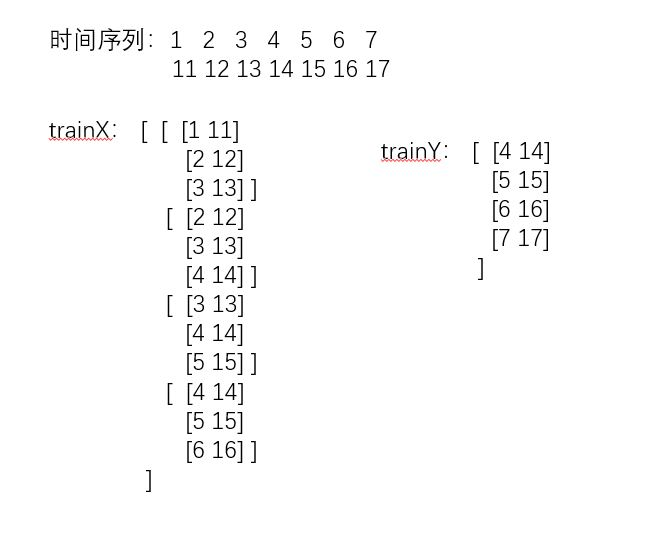from numpy import array
from numpy import hstack
from keras.models import Sequential
from keras.layers import LSTM
from keras.layers import Dense
# define input sequence
in_seq1 = array([10, 20, 30, 40, 50, 60, 70, 80, 90])
in_seq2 = array([15, 25, 35, 45, 55, 65, 75, 85, 95])
out_seq = array([in_seq1[i]+in_seq2[i] for i in range(len(in_seq1))])
# convert to [rows, columns] structure
in_seq1 = in_seq1.reshape((len(in_seq1), 1))
in_seq2 = in_seq2.reshape((len(in_seq2), 1))
out_seq = out_seq.reshape((len(out_seq), 1))
# horizontally stack columns
dataset = hstack((in_seq1, in_seq2, out_seq))
print(dataset)

'''
[[ 10  15  25]
[ 20  25  45]
[ 30  35  65]
[ 40  45  85]
[ 50  55 105]
[ 60  65 125]
[ 70  75 145]
[ 80  85 165]
[ 90  95 185]]
现在定为时间步长为3
举个例子，就是用
10 15
20 25
30 35
预测65
'''

# split a multivariate sequence into samples
def split_sequences(sequences, n_steps):
X, y = list(), list()
for i in range(len(sequences)):
end_ix = i + n_steps
if end_ix > len(sequences):
break
seq_x, seq_y = sequences[i:end_ix, :-1], sequences[end_ix-1, -1]   # 和单变量最大的不同在这里
X.append(seq_x)
y.append(seq_y)
return array(X), array(y)

n_steps = 3
X,y = split_sequences(dataset,n_steps)
print(X.shape,y.shape)
for i in range(len(X)):
print(X[i],y[i])

n_features = X.shape
'''
[[10 15]
[20 25]
[30 35]] 65
[[20 25]
[30 35]
[40 45]] 85
[[30 35]
[40 45]
[50 55]] 105
[[40 45]
[50 55]
[60 65]] 125
[[50 55]
[60 65]
[70 75]] 145
[[60 65]
[70 75]
[80 85]] 165
[[70 75]
[80 85]
[90 95]] 185
'''

model = Sequential()
model.fit(X,y,epochs=200,verbose=0)

#预测模型
x_input = array([[80, 85], [90, 95], [100, 105]])
x_input = x_input.reshape((1, n_steps, n_features))  # 转换成样本量+步长+特征的格式
yhat = model.predict(x_input, verbose=0)
print(yhat)                                          # 209.00851



多维多步的预测（使用前三步去预测后两步）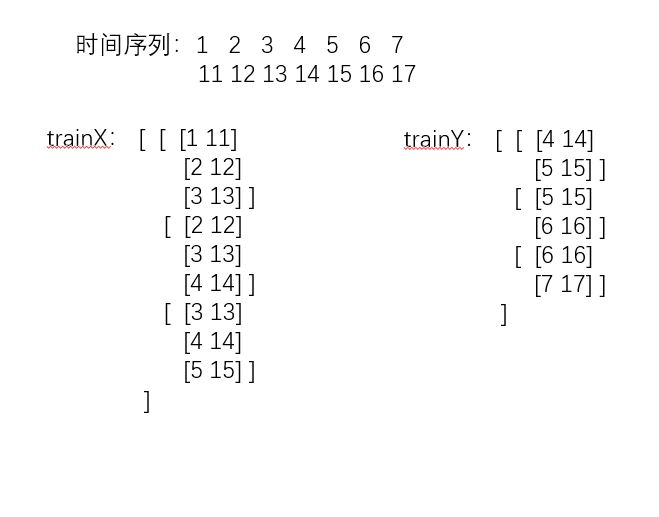from numpy import array
from numpy import hstack
from keras.models import Sequential
from keras.layers import LSTM
from keras.layers import Dense

# split a multivariate sequence into samples
def split_sequences(sequences, n_steps_in, n_steps_out):
X, y = list(), list()
for i in range(len(sequences)):
# find the end of this pattern
end_ix = i + n_steps_in
out_end_ix = end_ix + n_steps_out-1
# check if we are beyond the dataset
if out_end_ix > len(sequences):
break
# gather input and output parts of the pattern
seq_x, seq_y = sequences[i:end_ix, :-1], sequences[end_ix-1:out_end_ix, -1]
X.append(seq_x)
y.append(seq_y)
return array(X), array(y)

# define input sequence
in_seq1 = array([10, 20, 30, 40, 50, 60, 70, 80, 90])
in_seq2 = array([15, 25, 35, 45, 55, 65, 75, 85, 95])
out_seq = array([in_seq1[i]+in_seq2[i] for i in range(len(in_seq1))])
# convert to [rows, columns] structure
in_seq1 = in_seq1.reshape((len(in_seq1), 1))
in_seq2 = in_seq2.reshape((len(in_seq2), 1))
out_seq = out_seq.reshape((len(out_seq), 1))
# horizontally stack columns
dataset = hstack((in_seq1, in_seq2, out_seq))
# choose a number of time steps
n_steps_in, n_steps_out = 3, 2
# covert into input/output
X, y = split_sequences(dataset, n_steps_in, n_steps_out)
# the dataset knows the number of features, e.g. 2
n_features = X.shape
# define model
model = Sequential()
# fit model
model.fit(X, y, epochs=200, verbose=0)
# demonstrate prediction
x_input = array([[70, 75], [80, 85], [90, 95]])
x_input = x_input.reshape((1, n_steps_in, n_features))
yhat = model.predict(x_input, verbose=0)
print(yhat)
############################################################多变量，多对多

'''
[[10 15 25]
[20 25 45]
[30 35 65]] [[ 40  45  85]
[ 50  55 105]]-----y(输出为2*3维)
[[20 25 45]
[30 35 65]
[40 45 85]] [[ 50  55 105]
[ 60  65 125]]-----y
[[ 30  35  65]
[ 40  45  85]
[ 50  55 105]] [[ 60  65 125]
[ 70  75 145]]
[[ 40  45  85]
[ 50  55 105]
[ 60  65 125]] [[ 70  75 145]
[ 80  85 165]]
[[ 50  55 105]
[ 60  65 125]
[ 70  75 145]] [[ 80  85 165]
[ 90  95 185]]
'''

from numpy import array
from numpy import hstack
from keras.models import Sequential
from keras.layers import LSTM
from keras.layers import Dense
from keras.layers import RepeatVector
from keras.layers import TimeDistributed

# split a multivariate sequence into samples
def split_sequences(sequences, n_steps_in, n_steps_out):
X, y = list(), list()
for i in range(len(sequences)):
# find the end of this pattern
end_ix = i + n_steps_in
out_end_ix = end_ix + n_steps_out
# check if we are beyond the dataset
if out_end_ix > len(sequences):
break
# gather input and output parts of the pattern
seq_x, seq_y = sequences[i:end_ix, :], sequences[end_ix:out_end_ix, :]
X.append(seq_x)
y.append(seq_y)
return array(X), array(y)

# define input sequence
in_seq1 = array([10, 20, 30, 40, 50, 60, 70, 80, 90])
in_seq2 = array([15, 25, 35, 45, 55, 65, 75, 85, 95])
out_seq = array([in_seq1[i]+in_seq2[i] for i in range(len(in_seq1))])
# convert to [rows, columns] structure
in_seq1 = in_seq1.reshape((len(in_seq1), 1))
in_seq2 = in_seq2.reshape((len(in_seq2), 1))
out_seq = out_seq.reshape((len(out_seq), 1))
# horizontally stack columns
dataset = hstack((in_seq1, in_seq2, out_seq))
# choose a number of time steps
n_steps_in, n_steps_out = 3, 2
# covert into input/output
X, y = split_sequences(dataset, n_steps_in, n_steps_out)
# the dataset knows the number of features, e.g. 2
n_features = X.shape
# define model
model = Sequential()
'''
这里输入3D为（样本量，return_sequences数量（=n_steps_out）,200）
输出为（样本量，return_sequences数量（=n_steps_out），n_features）
就是每个输出是（3,2)维度的
'''
# fit model
model.fit(X, y, epochs=300, verbose=0)
# demonstrate prediction
x_input = array([[60, 65, 125], [70, 75, 145], [80, 85, 165]])
x_input = x_input.reshape((1, n_steps_in, n_features))
yhat = model.predict(x_input, verbose=0)
print(yhat)

'''
总结：注意的点：
最大不同在DENSE的输出层，其实在多步骤的时候什么时候用n_featues,什么时候用n_steps还是看y的格式鸭



展开全文• 时间序列预测8种常用方法简介，包括朴素预测法、简单平均法、移动平均法、简单指数平滑法、霍尔特(Holt)线性趋势法、Holt-Winter方法、AMRIA。
时间序列预测的7种方法
1. 朴素预测法（Naive Forecast)
如果数据集在一段时间内都很稳定，我们想预测第二天的价格，可以取前面一天的价格，预测第二天的值。这种假设第一个预测点和上一个观察点相等的预测方法就叫朴素法，即 $y_{t+1} = y_t$2. 简单平均法(Simple Average）
这种方法预测的期望值等于所有先前观测点的平均值，称为简单平均法。。
物品价格会随机上涨和下跌，平均价格会保持一致。我们经常会遇到一些数据集，虽然在一定时期内出现小幅变动，但每个时间段的平均值确实保持不变。这种情况下，我们可以认为第二天的价格大致和过去的平均价格值一致。这种将预期值等同于之前所有观测点的平均值的预测方法就叫简单平均法。即
$\widehat{y}_{x+1} =1/x \sum\nolimits_{i=1}^xy_{i}\,.$
由图可见，这种方法并没有提高结果的准确度。因此，可以推断出，当每个时间段的平均值保持不变时，这种方法效果最好。3. 移动平均法(Moving Average）
移动平均法也叫滑动平均法，取前面n个点的平均值作为预测值从图表中我们可以推断出，过去的观测值在这段时间里有很大幅度的上涨。如果使用简单平均法，我们必须使用所有历史数据的平均值，但是使用所有数据得出的结果并不正确。
因此，作为改进，我们只取最近几个时期的平均价格。显然，这里的想法是，只有最近的价值才重要。这种利用时间窗计算平均值的预测技术称为移动平均法。移动平均值的计算有时包括一个大小为n的“滑动窗口”。
计算移动平均值涉及到一个有时被称为“滑动窗口”的大小值p。使用简单的移动平均模型，我们可以根据之前数值的固定有限数p的平均值预测某个时序中的下一个值。这样，对于所有的 i>p
利用一个简单的移动平均模型，我们预测一个时间序列中的下一个值是基于先前值的固定有限个数“p”的平均值。因此，对于所有i>p4. 加权移动平均(Weighted Moving Average)
加权移动平均法是对移动平均法的一个改进。在如上所述的移动平均法中，我们对过去的n个观测值进行了同等的加权。但我们可能会遇到这样的情况：过去“n”的每一个观察结果都会以不同的方式影响预测。这种对过去观测值进行不同加权的技术称为加权移动平均法。
加权移动平均法其实还是一种移动平均法，只是“滑动窗口期”内的值被赋予不同的权重，通常来讲，最近时间点的值越重要。即
这种方法并非选择一个窗口期的值，而是需要一列权重值（相加后为1）。例如，如果我们选择[0.40, 0.25, 0.20, 0.15]作为权值，我们会为最近的4个时间点分别赋给40%，25%，20%和15%的权重。5. 简单指数平滑法 (Simple Exponential Smoothing)
我们注意到简单平均法和加权移动平均法在选取时间点的思路上存在较大的差异：简单平均法将过去数据一个不漏地全部加以同等利用；移动平均法则不考虑较远期的数据，并在加权移动平均法中给予近期更大的权重。我们就需要在这两种方法之间取一个折中的方法，在将所有数据考虑在内的同时也能给数据赋予不同非权重。
指数平滑法相比更早时期内的观测值，越近的观测值会被赋予更大的权重，而时间越久远的权重越小。它通过加权平均值计算出预测值，其中权重随着观测值从早期到晚期的变化呈指数级下降，最小的权重和最早的观测值相关：其中0≤α≤1是平滑参数。
对时间点T+1的预测值是时序y1,…,yT的所有观测值的加权平均数。权重下降的速率由参数α控制，
因此，它可以写为：简单指数平滑法预测公式为：
$y_{t+1}'=ay_t+(1-a)yt'$ 式中，
$y_{t+1}'$ : t+1期的预测值，即本期（t期）的平滑值St ；
$yt$: t期的实际值；
$yt'$ : t期的预测值，即上期的平滑值St-1 。
该公式又可以写作：$y_{t+1}'=y_t'+a(y_t- y_t')$。
可见，下期预测值又是本期预测值与以a为折扣的本期实际值与预测值误差之和。6. 霍尔特线性趋势法
Holts线性趋势模型，霍尔特线性趋势法，该方法考虑了数据集的趋势，即序列的增加或减少性质。
尽管这些方法中的每一种都可以应用趋势：简单平均法会假设最后两点之间的趋势保持不变，或者我们可以平均所有点之间的所有斜率以获得平均趋势，使用移动趋势平均值或应用指数平滑。
但我们需要一种无需任何假设就能准确绘制趋势图的方法。这种考虑数据集趋势的方法称为霍尔特线性趋势法，或者霍尔特指数平滑法。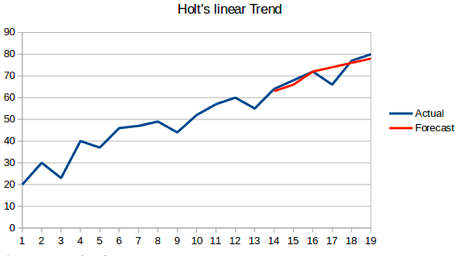7. Holt-Winters方法（三次指数平滑）
霍尔特-温特（Holt-Winters）方法，有的地方也叫三次指数平滑法。Holt-Winters 方法在 Holt模型基础上引入了 Winters 周期项（也叫做季节项），可以用来处理月度数据（周期 12）、季度数据（周期 4）、星期数据（周期 7）等时间序列中的固定周期的波动行为。引入多个 Winters 项还可以处理多种周期并存的情况。
当一个序列在每个固定的时间间隔中都出现某种重复的模式，就称之具有季节性特征，而这样的一个时间间隔称为一个季节性特征。
例如酒店的预订量在周末较高，工作日较低，并且每年都在增加， 表明存在一个一周的季节性和增长趋势。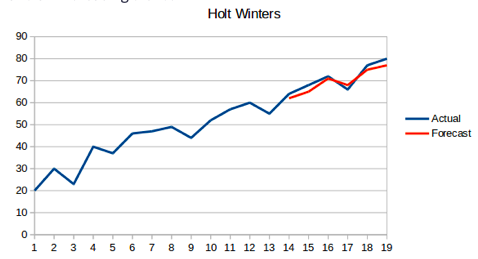8. ARIMA
其他的预测方法，如自回归模型 AR§、移动平均模型 MA(q)、自回归移动平均模型 ARMA(p,q)、自回归差分移动平均模型 ARIMA(p,d,q), 可以说前三种都是 ARIMA(p,d,q)模型的特殊形式。
其中最经典的就是ARIMA模型：
ARIMA模型（英语：Autoregressive Integrated Moving Average model），差分整合移动平均自回归模型，又称整合移动平均自回归模型（移动也可称作滑动），是时间序列预测分析方法之一。ARIMA(p，d，q)中，AR是“自回归”，p为自回归项数；MA为“滑动平均”，q为滑动平均项数，d为使之成为平稳序列所做的差分次数（阶数）。“差分”一词虽未出现在ARIMA的英文名称中，却是关键步骤。
关于ARIMA的内容很多，后续会另外发表笔记总结相关知识，以及这几种方法的实例比较。

部分参考资料
Forecasting: Principles and Practice： https://otexts.com/fpp2/holt-winters.html
加权移动平均法：https://wiki.mbalib.com/wiki/%E7%A7%BB%E5%8A%A8%E5%B9%B3%E5%9D%87%E6%B3%95#.E4.B8.80.E3.80.81.E7.AE.80.E5.8D.95.E7.A7.BB.E5.8A.A8.E5.B9.B3.E5.9D.87.E6.B3.95
趋势的预测：霍尔特指数平滑法 https://zhuanlan.zhihu.com/p/164919931)
指数平滑法 https://wiki.mbalib.com/wiki/指数平滑法
二次指数平滑法：https://wiki.mbalib.com/wiki/二次指数平滑法


展开全文• 我们将首先介绍和讨论自相关，平稳性和季节性的概念，并继续应用最常用的时间序列预测方法之一，称为ARIMA。 介绍 时间序列提供了预测未来价值的机会。基于以前的价值观，可以使用时间序列来预测经济，天气和...
Python 3中使用ARIMA进行时间序列预测的指南

在本教程中，我们将提供可靠的时间序列预测。我们将首先介绍和讨论自相关，平稳性和季节性的概念，并继续应用最常用的时间序列预测方法之一，称为ARIMA。

介绍

时间序列提供了预测未来价值的机会。 基于以前的价值观，可以使用时间序列来预测经济，天气和能力规划的趋势，其中仅举几例。 时间序列数据的具体属性意味着通常需要专门的统计方法。

在本教程中，我们将针对时间序列产生可靠的预测。 我们将首先介绍和讨论自相关，平稳性和季节性的概念，并继续应用最常用的时间序列预测方法之一，称为ARIMA。

用于建模和预测时间序列未来点的Python中的一种方法被称为SARIMAX ，其代表具有eXogenous回归的季节性自动反馈集成移动平均值 。 在这里，我们将主要关注ARIMA组件，该组件用于适应时间序列数据，以更好地了解和预测时间序列中的未来点。

时间序列预测——ARIMA（差分自回归移动平均模型）

ARIMA（p，d，q）中，AR是"自回归"，p为自回归项数；I为差分，d为使之成为平稳序列所做的差分次数（阶数）；MA为"滑动平均"，q为滑动平均项数，。ACF自相关系数能决定q的取值，PACF偏自相关系数能够决定q的取值。ARIMA原理：将非平稳时间序列转化为平稳时间序列然后将因变量仅对它的滞后值以及随机误差项的现值和滞后值进行回归所建立的模型

基本解释：

自回归模型（AR）

描述当前值与历史值之间的关系，用变量自身的历史时间数据对自身进行预测
自回归模型必须满足平稳性的要求
必须具有自相关性，自相关系数小于0.5则不适用
p阶自回归过程的公式定义：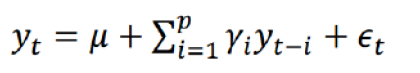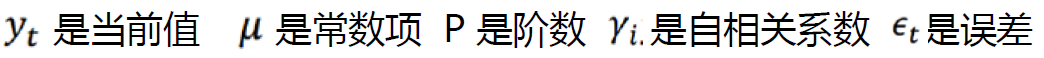，y t-i 为前几天的值

PACF，偏自相关函数（决定p值），剔除了中间k-1个随机变量x(t-1)、x(t-2)、……、x(t-k+1)的干扰之后x(t-k)对x(t)影响的相关程度。

移动平均模型（MA）

移动平均模型关注的是自回归模型中的误差项的累加，移动平均法能有效地消除预测中的随机波动
q阶自回归过程的公式定义：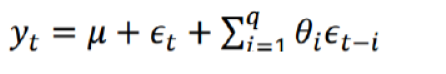ACF，自相关函数（决定q值）反映了同一序列在不同时序的取值之间的相关性。x(t)同时还会受到中间k-1个随机变量x(t-1)、x(t-2)、……、x(t-k+1)的影响而这k-1个随机变量又都和x(t-k)具有相关关系，所 以自相关系数p(k)里实际掺杂了其他变量对x(t)与x(t-k)的影响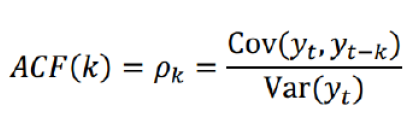ARIMA(p，d，q)阶数确定：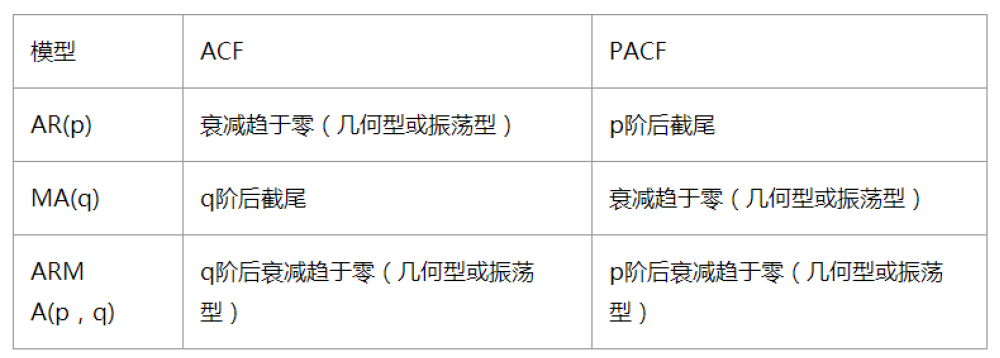截尾：落在置信区间内（95%的点都符合该规则）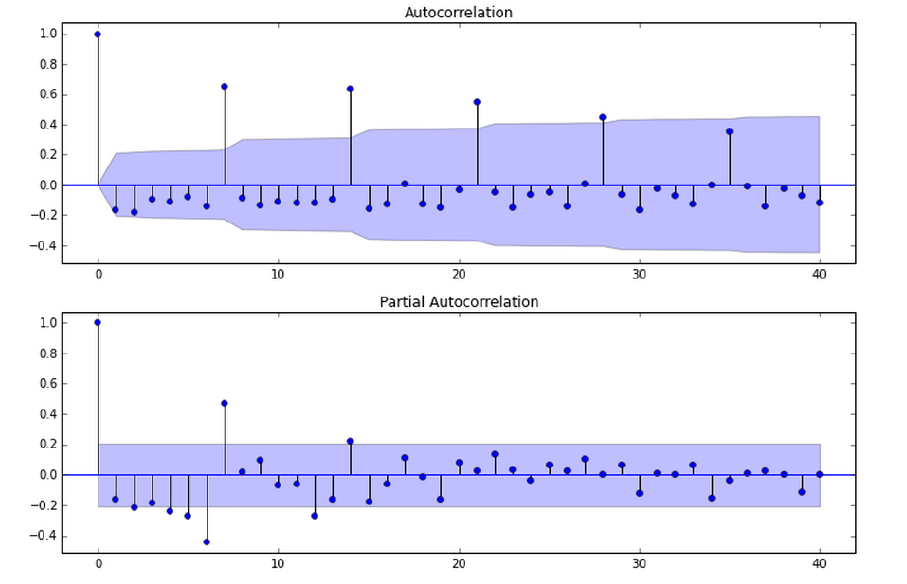acf和pacf图

平稳性要求：平稳性就是要求经由样本时间序列所得到的拟合曲线在未来的一段期间内仍能顺着现有的形态“惯性”地延续下去

平稳性要求序列的均值和方差不发生明显变化。

具体分为严平稳与弱平稳：    严平稳：严平稳表示的分布不随时间的改变而改变。
如：白噪声（正态），无论怎么取，都是期望为0，方差为1    弱平稳：期望与相关系数（依赖性）不变
未来某时刻的t的值Xt就要依赖于它的过去信息，所以需要依赖性

因为实际生活中我们拿到的数据基本都是弱平稳数据，为了保证ARIMA模型的要求，我们需要对数据进行差分,以求数据变的平稳。

模型评估：

AIC:赤池信息准则（AkaikeInformation Criterion，AIC）
???=2?−2ln(?)
BIC:贝叶斯信息准则（Bayesian Information Criterion，BIC）
???=????−2ln(?)
k为模型参数个数，n为样本数量，L为似然函数

模型残差检验：

ARIMA模型的残差是否是平均值为0且方差为常数的正态分布
QQ图：线性即正态分布
先决条件

本指南将介绍如何在本地桌面或远程服务器上进行时间序列分析。 使用大型数据集可能是内存密集型的，所以在这两种情况下，计算机将至少需要2GB的内存来执行本指南中的一些计算。

要充分利用本教程，熟悉时间序列和统计信息可能会有所帮助。

对于本教程，我们将使用Jupyter Notebook来处理数据。 如果您还没有，您应该遵循我们的教程安装和设置Jupyter Notebook for Python 3 。

第1步 - 安装软件包

为了建立我们的时间序列预测环境，我们先进入本地编程环境或基于服务器的编程环境：

cd environments


. my_env/bin/activate

从这里，我们为我们的项目创建一个新的目录。 我们称之为ARIMA ，然后进入目录。 如果您将项目称为不同名称，请务必在整个指南中将您的名称替换为ARIMA

mkdir ARIMA
cd ARIMA


本教程将需要warnings ， itertools ， itertools ， numpy ， matplotlib和statsmodels库。 warnings和itertools库包含在标准Python库集中，因此您不需要安装它们。

像其他Python包一样，我们可以用pip安装这些要求。
我们现在可以安装pandas ， statsmodels和数据绘图包matplotlib 。 它们的依赖也将被安装：

pip install pandas numpy statsmodels matplotlib

在这一点上，我们现在设置为开始使用已安装的软件包。

第2步 - 导入包并加载数据

要开始使用我们的数据，我们将启动Jupyter Notebook：

jupyter notebook

要创建新的笔记本文件，请从右上角的下拉菜单中选择新建 > Python 3 ：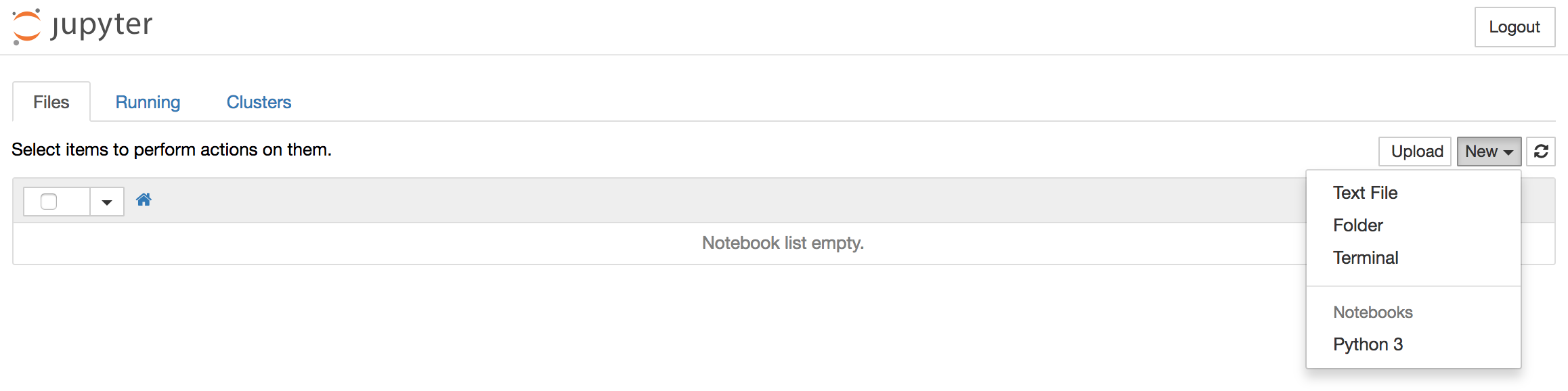这将打开一个笔记本。

最好的做法是，从笔记本电脑的顶部导入需要的库：

import warnings
import itertools
import pandas as pd
import numpy as np
import statsmodels.api as sm
import matplotlib.pyplot as plt
plt.style.use('fivethirtyeight')

我们还为我们的地块定义了一个matplotlib风格 。

我们将使用一个名为“来自美国夏威夷Mauna Loa天文台的连续空气样本的大气二氧化碳”的数据集，该数据集从1958年3月至2001年12月期间收集了二氧化碳样本。我们可以提供如下数据：

data = sm.datasets.co2.load_pandas()
y = data.data

让我们稍后再进行一些数据处理。 每周数据可能很棘手，因为它是一个很短的时间，所以让我们使用每月平均值。 我们将使用resample函数进行转换。 为了简单起见，我们还可以使用fillna()函数来确保我们的时间序列中没有缺少值。

# The 'MS' string groups the data in buckets by start of the month
y = y['co2'].resample('MS').mean()

# The term bfill means that we use the value before filling in missing values
y = y.fillna(y.bfill())

print(y)

Outputco2
1958-03-01  316.100000
1958-04-01  317.200000
1958-05-01  317.433333
...
2001-11-01  369.375000
2001-12-01  371.020000

我们来探索这个时间序列e作为数据可视化：

y.plot(figsize=(15, 6))
plt.show()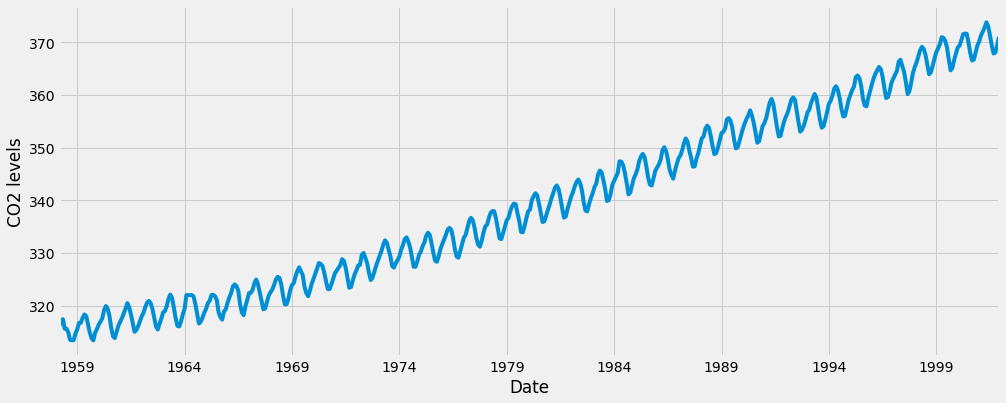当我们绘制数据时，会出现一些可区分的模式。 时间序列具有明显的季节性格局，总体呈上升趋势。

要了解有关时间序列预处理的更多信息，请参阅“ 使用Python 3进行时间序列可视化的指南 ”，其中上面的步骤将更详细地描述。

现在我们已经转换和探索了我们的数据，接下来我们继续使用ARIMA进行时间序列预测。

第3步 - ARIMA时间序列模型

时间序列预测中最常用的方法之一就是被称为ARIMA模型，它代表了A utoreg R essive综合M oving A版本 。 ARIMA是可以适应时间序列数据的模型，以便更好地了解或预测系列中的未来点。

有三个不同的整数（ p ， d ， q ）用于参数化ARIMA模型。 因此，ARIMA模型用符号ARIMA(p, d, q) 。 这三个参数共计数据集中的季节性，趋势和噪音：

p是模型的自回归部分。 它允许我们将过去价值观的影响纳入我们的模型。 直观地说，这将是类似的，表示如果过去3天已经变暖，明天可能会变暖。
d是模型的集成部分。 这包括模型中包含差异量（即从当前值减去的过去时间点的数量）以适用于时间序列的术语。 直观地说，这将类似于说如果过去三天的温度差异非常小，明天可能会有相同的温度。
q是模型的移动平均部分。 这允许我们将模型的误差设置为过去以前时间点观察到的误差值的线性组合。
在处理季节性影响时，我们利用季节性 ARIMA，表示为ARIMA(p,d,q)(P,D,Q)s 。 这里， (p, d, q)是上述非季节性参数，而(P, D, Q)遵循相同的定义，但适用于时间序列的季节分量。 术语s是时间序列的周期（季度为4 ，年度为12 ，等等）。

由于所涉及的多个调整参数，季节性ARIMA方法可能会令人望而生畏。 在下一节中，我们将介绍如何自动化识别季节性ARIMA时间序列模型的最优参数集的过程。

第4步 - ARIMA时间序列模型的参数选择

当考虑使用季节性ARIMA模型拟合时间序列数据时，我们的第一个目标是找到优化感兴趣度量的ARIMA(p,d,q)(P,D,Q)s的值。 实现这一目标有许多指导方针和最佳实践，但ARIMA模型的正确参数化可能是一个需要领域专长和时间的艰苦的手工过程。 其他统计编程语言（如R提供了自动化的方法来解决这个问题 ，但尚未被移植到Python中。 在本节中，我们将通过编写Python代码来编程选择ARIMA(p,d,q)(P,D,Q)s时间序列模型的最优参数值来解决此问题。

非季节性参数：p，d，q

季节参数：P、D、Q

s：时间序列的周期，年周期s=12

我们将使用“网格搜索”来迭代地探索参数的不同组合。 对于参数的每个组合，我们使用statsmodels模块的SARIMAX()函数拟合一个新的季节性ARIMA模型，并评估其整体质量。 一旦我们探索了参数的整个范围，我们的最佳参数集将是我们感兴趣的标准产生最佳性能的参数。 我们开始生成我们希望评估的各种参数组合：

# Define the p, d and q parameters to take any value between 0 and 2
p = d = q = range(0, 2)

# Generate all different combinations of p, q and q triplets
pdq = list(itertools.product(p, d, q))

# Generate all different combinations of seasonal p, q and q triplets
seasonal_pdq = [(x, x, x, 12) for x in list(itertools.product(p, d, q))]

print('Examples of parameter combinations for Seasonal ARIMA...')
print('SARIMAX: {} x {}'.format(pdq, seasonal_pdq))
print('SARIMAX: {} x {}'.format(pdq, seasonal_pdq))
print('SARIMAX: {} x {}'.format(pdq, seasonal_pdq))
print('SARIMAX: {} x {}'.format(pdq, seasonal_pdq))

Output
Examples of parameter combinations for Seasonal ARIMA...
SARIMAX: (0, 0, 1) x (0, 0, 1, 12)
SARIMAX: (0, 0, 1) x (0, 1, 0, 12)
SARIMAX: (0, 1, 0) x (0, 1, 1, 12)
SARIMAX: (0, 1, 0) x (1, 0, 0, 12)

我们现在可以使用上面定义的参数三元组来自动化不同组合对ARIMA模型进行培训和评估的过程。 在统计和机器学习中，这个过程被称为模型选择的网格搜索（或超参数优化）。

在评估和比较配备不同参数的统计模型时，可以根据数据的适合性或准确预测未来数据点的能力，对每个参数进行排序。 我们将使用AIC （Akaike信息标准）值，该值通过使用statsmodels安装的ARIMA型号方便地返回。 AIC衡量模型如何适应数据，同时考虑到模型的整体复杂性。 在使用大量功能的情况下，适合数据的模型将被赋予比使用较少特征以获得相同的适合度的模型更大的AIC得分。 因此，我们有兴趣找到产生最低AIC值的模型。

下面的代码块通过参数的组合来迭代，并使用SARIMAX函数来适应相应的季节性ARIMA模型。 这里， order参数指定(p, d, q)参数，而seasonal_order参数指定季节性ARIMA模型的(P, D, Q, S)季节分量。 在安装每个SARIMAX()模型后，代码打印出其各自的AIC得分。

warnings.filterwarnings("ignore") # specify to ignore warning messages

for param in pdq:
for param_seasonal in seasonal_pdq:
try:
mod = sm.tsa.statespace.SARIMAX(y,
order=param,
seasonal_order=param_seasonal,
enforce_stationarity=False,
enforce_invertibility=False)

results = mod.fit()

print('ARIMA{}x{}12 - AIC:{}'.format(param, param_seasonal, results.aic))
except:
continue

由于某些参数组合可能导致数字错误指定，因此我们明确禁用警告消息，以避免警告消息过载。 这些错误指定也可能导致错误并引发异常，因此我们确保捕获这些异常并忽略导致这些问题的参数组合。

上面的代码应该产生以下结果，这可能需要一些时间：

Output
SARIMAX(0, 0, 0)x(0, 0, 1, 12) - AIC:6787.3436240402125
SARIMAX(0, 0, 0)x(0, 1, 1, 12) - AIC:1596.711172764114
SARIMAX(0, 0, 0)x(1, 0, 0, 12) - AIC:1058.9388921320026
SARIMAX(0, 0, 0)x(1, 0, 1, 12) - AIC:1056.2878315690562
SARIMAX(0, 0, 0)x(1, 1, 0, 12) - AIC:1361.6578978064144
SARIMAX(0, 0, 0)x(1, 1, 1, 12) - AIC:1044.7647912940095
...
...
...
SARIMAX(1, 1, 1)x(1, 0, 0, 12) - AIC:576.8647112294245
SARIMAX(1, 1, 1)x(1, 0, 1, 12) - AIC:327.9049123596742
SARIMAX(1, 1, 1)x(1, 1, 0, 12) - AIC:444.12436865161305
SARIMAX(1, 1, 1)x(1, 1, 1, 12) - AIC:277.7801413828764

我们的代码的输出表明， SARIMAX(1, 1, 1)x(1, 1, 1, 12)产生最低的AIC值为277.78。 因此，我们认为这是我们考虑过的所有模型中的最佳选择。

第5步 - 安装ARIMA时间序列模型

使用网格搜索，我们已经确定了为我们的时间序列数据生成最佳拟合模型的参数集。 我们可以更深入地分析这个特定的模型。

我们首先将最佳参数值插入到新的SARIMAX模型中：

mod = sm.tsa.statespace.SARIMAX(y,
order=(1, 1, 1),
seasonal_order=(1, 1, 1, 12),
enforce_stationarity=False,
enforce_invertibility=False)

results = mod.fit()

print(results.summary().tables) #详细输出，results.summary()可以输出全部的模型计算参数表

Output
==============================================================================
coef    std err          z      P>|z|      [0.025      0.975]
------------------------------------------------------------------------------
ar.L1          0.3182      0.092      3.443      0.001       0.137       0.499
ma.L1         -0.6255      0.077     -8.165      0.000      -0.776      -0.475
ar.S.L12       0.0010      0.001      1.732      0.083      -0.000       0.002
ma.S.L12      -0.8769      0.026    -33.811      0.000      -0.928      -0.826
sigma2         0.0972      0.004     22.634      0.000       0.089       0.106
==============================================================================

由SARIMAX的输出产生的SARIMAX返回大量的信息，但是我们将注意力集中在系数表上。 coef列显示每个特征的重量（即重要性）以及每个特征如何影响时间序列。 P>|z| 列通知我们每个特征重量的意义。 这里，每个重量的p值都低于或接近0.05 ，所以在我们的模型中保留所有权重是合理的。

在 fit 季节性ARIMA模型（以及任何其他模型）的情况下，运行模型诊断是非常重要的，以确保没有违反模型的假设。 plot_diagnostics对象允许我们快速生成模型诊断并调查任何异常行为。

results.plot_diagnostics(figsize=(15, 12))
plt.show()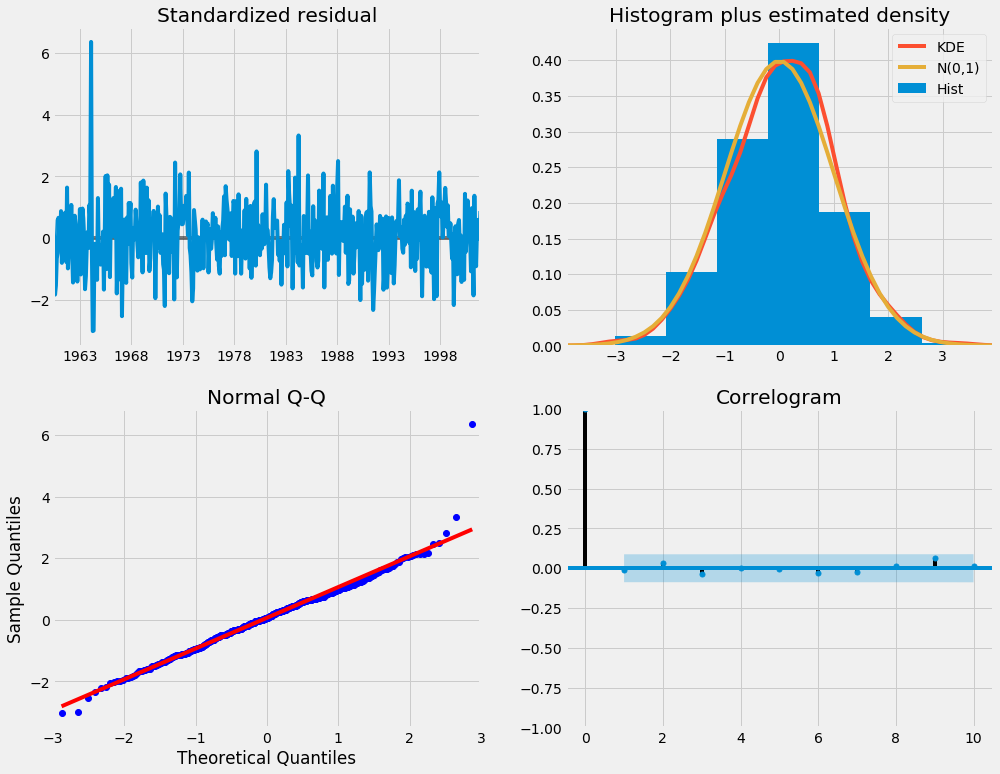我们的主要关切是确保我们的模型的残差是不相关的，并且平均分布为零。 如果季节性ARIMA模型不能满足这些特性，这是一个很好的迹象，可以进一步改善。

残差在数理统计中是指实际观察值与估计值（拟合值）之间的差。“残差”蕴含了有关模型基本假设的重要信息。如果回归模型正确的话， 我们可以将残差看作误差的观测值。 它应符合模型的假设条件，且具有误差的一些性质。利用残差所提供的信息，来考察模型假设的合理性及数据的可靠性称为残差分析

在这种情况下，我们的模型诊断表明，模型残差正常分布如下：

在右上图中，我们看到红色KDE线与N(0,1)行（其中N(0,1) ）是正态分布的标准符号，平均值0 ，标准偏差为1 ） 。 这是残留物正常分布的良好指示。

左下角的qq图显示，残差（蓝点）的有序分布遵循采用N(0, 1)的标准正态分布采样的线性趋势。 同样，这是残留物正常分布的强烈指示。

随着时间的推移（左上图）的残差不会显示任何明显的季节性，似乎是白噪声。 这通过右下角的自相关（即相关图）来证实，这表明时间序列残差与其本身的滞后值具有低相关性。

这些观察结果使我们得出结论，我们的模型选择了令人满意，可以帮助我们了解我们的时间序列数据和预测未来价值。

虽然我们有一个令人满意的结果，我们的季节性ARIMA模型的一些参数可以改变，以改善我们的模型拟合。 例如，我们的网格搜索只考虑了一组受限制的参数组合，所以如果我们拓宽网格搜索，我们可能会找到更好的模型。

第6步 - 验证预测

我们已经获得了我们时间序列的模型，现在可以用来产生预测。 我们首先将预测值与时间序列的实际值进行比较，这将有助于我们了解我们的预测的准确性。 get_prediction()和conf_int()属性允许我们获得时间序列预测的值和相关的置信区间。

pred = results.get_prediction(start=pd.to_datetime('1998-01-01'), dynamic=False)#预测值
pred_ci = pred.conf_int()#置信区间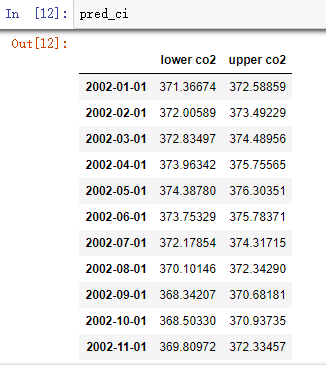上述规定需要从1998年1月开始进行预测。

dynamic=False参数确保我们每个点的预测将使用之前的所有历史观测。

我们可以绘制二氧化碳时间序列的实际值和预测值，以评估我们做得如何。 注意我们如何在时间序列的末尾放大日期索引。

ax = y['1990':].plot(label='observed')

ax.fill_between(pred_ci.index,
pred_ci.iloc[:, 0],
pred_ci.iloc[:, 1], color='k', alpha=.2)
#图形填充fill fill_between，参考网址：
#https://www.cnblogs.com/gengyi/p/9416845.html

ax.set_xlabel('Date')
ax.set_ylabel('CO2 Levels')
plt.legend()

plt.show()

pred.predicted_mean 可以得到预测均值，就是置信区间的上下加和除以2

函数间区域填充 fill_between用法：

plt.fill_between( x, y1, y2=0, where=None, interpolate=False, step=None, hold=None,data=None,  **kwarg)

x - array( length N) 定义曲线的 x 坐标

y1 - array( length N ) or scalar 定义第一条曲线的 y 坐标

y2 - array( length N )  or scalar 定义第二条曲线的 y 坐标

where - array of bool (length N), optional, default: None 排除一些（垂直）区域被填充。注：我理解的垂直区域，但帮助文档上写的是horizontal regions

也可简单地描述为：

plt.fill_between(x，y1，y2，where=条件表达式, color=颜色，alpha=透明度)" where = " 可以省略，直接写条件表达式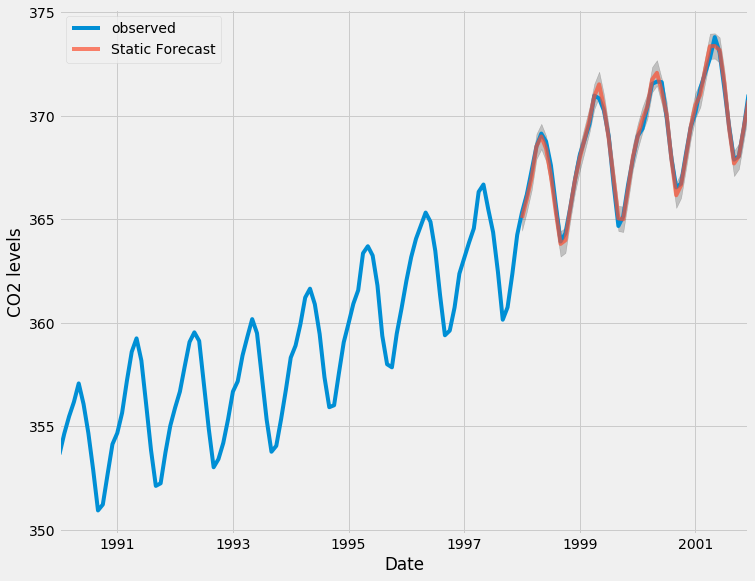总体而言，我们的预测与真实价值保持一致，呈现总体增长趋势。

量化预测的准确性

这是很有必要的。 我们将使用MSE（均方误差，也就是方差），它总结了我们的预测的平均误差。 对于每个预测值，我们计算其到真实值的距离并对结果求平方。 结果需要平方，以便当我们计算总体平均值时，正/负差异不会相互抵消。

y_forecasted = pred.predicted_mean
y_truth = y['1998-01-01':]

# Compute the mean square error
mse = ((y_forecasted - y_truth) ** 2).mean()
print('The Mean Squared Error of our forecasts is {}'.format(round(mse, 2)))

Output
The Mean Squared Error of our forecasts is 0.07

我们前进一步预测的MSE值为0.07 ，这是接近0的非常低的值。MSE=0是预测情况将是完美的精度预测参数的观测值，但是在理想的场景下通常不可能。

然而，使用动态预测(dynamic=True)可以获得更好地表达我们的真实预测能力。 在这种情况下，我们只使用时间序列中的信息到某一点，之后，使用先前预测时间点的值生成预测。

在下面的代码块中，我们指定从1998年1月起开始计算动态预测和置信区间。

pred_dynamic = results.get_prediction(start=pd.to_datetime('1998-01-01'), dynamic=True, full_results=True)
pred_dynamic_ci = pred_dynamic.conf_int()

绘制时间序列的观测值和预测值，我们看到即使使用动态预测，总体预测也是准确的。 所有预测值（红线）与地面真相（蓝线）相当吻合，并且在我们预测的置信区间内。

ax = y['1990':].plot(label='observed', figsize=(20, 15))
pred_dynamic.predicted_mean.plot(label='Dynamic Forecast', ax=ax)

ax.fill_between(pred_dynamic_ci.index,
pred_dynamic_ci.iloc[:, 0],
pred_dynamic_ci.iloc[:, 1], color='k', alpha=.25)

ax.fill_betweenx(ax.get_ylim(), pd.to_datetime('1998-01-01'), y.index[-1],
alpha=.1, zorder=-1)

ax.set_xlabel('Date')
ax.set_ylabel('CO2 Levels')

plt.legend()
plt.show()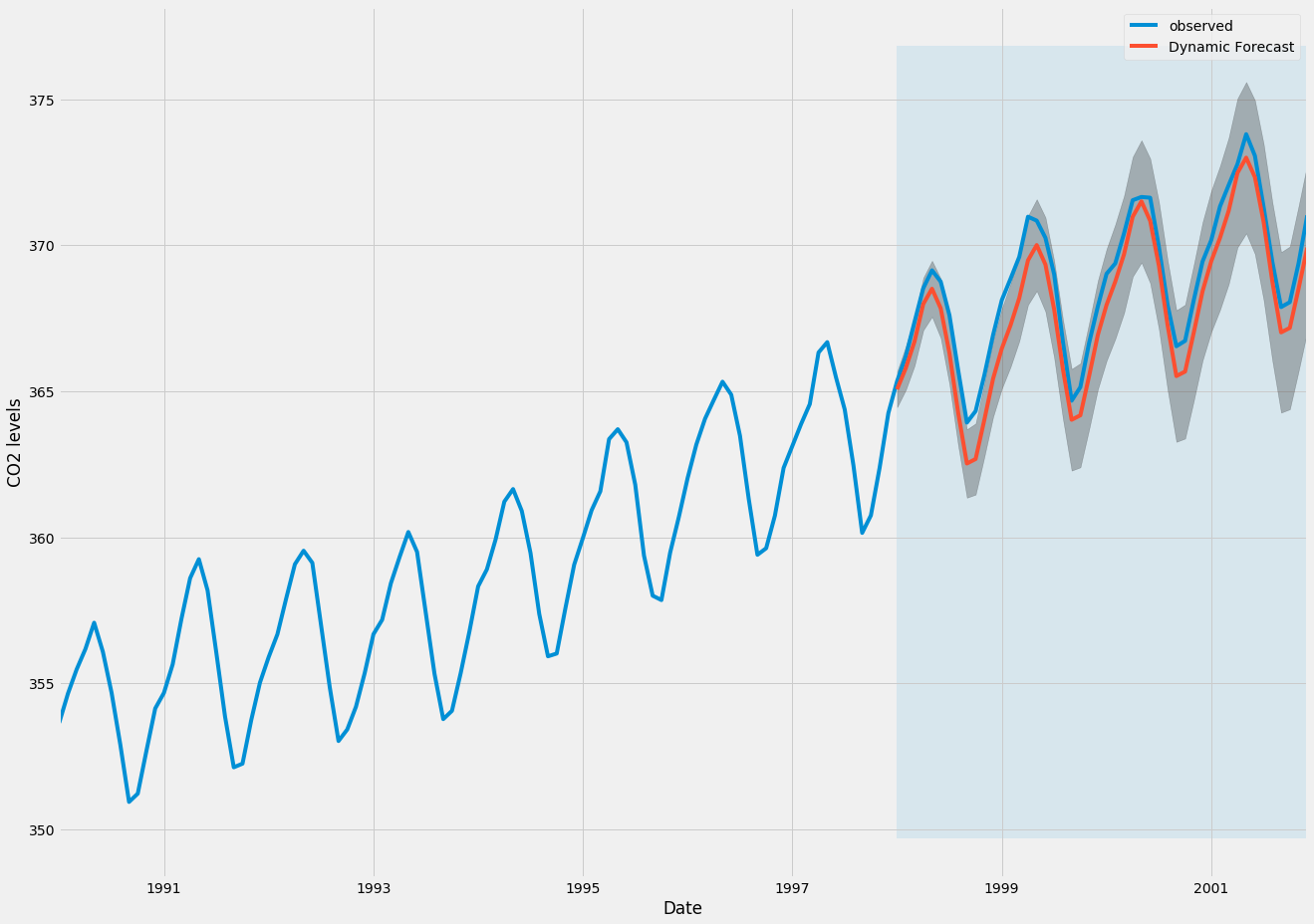我们再次通过计算MSE量化我们预测的预测性能：

# Extract the predicted and true values of our time-series
y_forecasted = pred_dynamic.predicted_mean
y_truth = y['1998-01-01':]

# Compute the mean square error
mse = ((y_forecasted - y_truth) ** 2).mean()
print('The Mean Squared Error of our forecasts is {}'.format(round(mse, 2)))

OutputThe Mean Squared Error of our forecasts is 1.01

从动态预测获得的预测值产生1.01的MSE。 这比前进一步略高，这是意料之中的，因为我们依赖于时间序列中较少的历史数据。

前进一步和动态预测都证实了这个时间序列模型是有效的。 然而，关于时间序列预测的大部分兴趣是能够及时预测未来价值观。

第7步 - 生成和可视化预测

在本教程的最后一步，我们将介绍如何利用季节性ARIMA时间序列模型来预测未来的价值。 我们的时间序列对象的

get_forecast()属性可以计算预先指定数量的步骤的预测值。

# Get forecast 500 steps ahead in future
pred_uc = results.get_forecast(steps=500)

# Get confidence intervals of forecasts
pred_ci = pred_uc.conf_int()

我们可以使用此代码的输出绘制其未来值的时间序列和预测。

ax = y.plot(label='observed', figsize=(20, 15))
pred_uc.predicted_mean.plot(ax=ax, label='Forecast')
ax.fill_between(pred_ci.index,
pred_ci.iloc[:, 0],
pred_ci.iloc[:, 1], color='k', alpha=.25)
ax.set_xlabel('Date')
ax.set_ylabel('CO2 Levels')

plt.legend()
plt.show()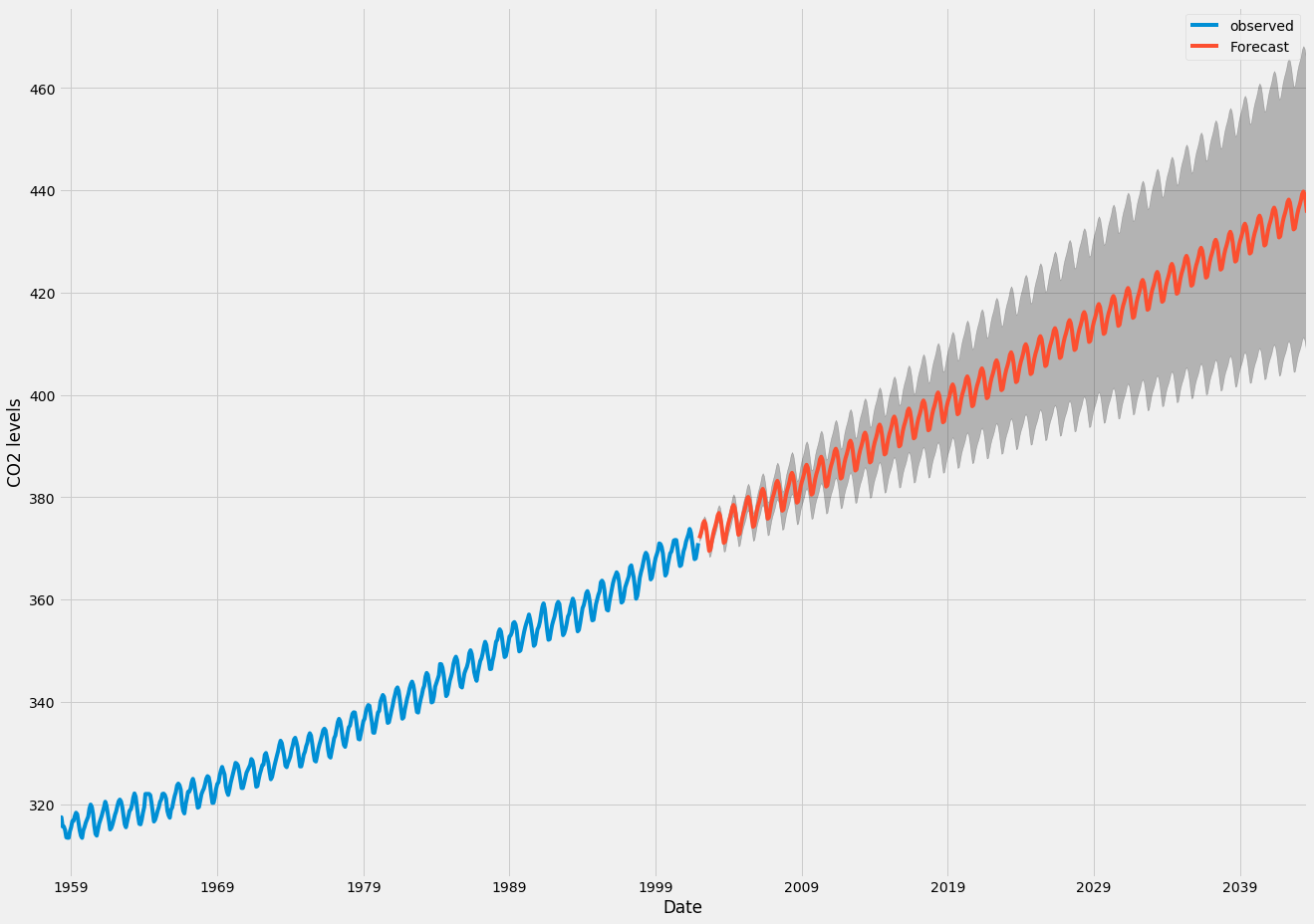现在可以使用我们生成的预测和相关的置信区间来进一步了解时间序列并预见预期。 我们的预测显示，时间序列预计将继续稳步增长。

随着我们对未来的进一步预测，我们对自己的价值观信心愈来愈自然。 这反映在我们的模型产生的置信区间，随着我们进一步走向未来，这个模型越来越大。

结论

在本教程中，我们描述了如何在Python中实现季节性ARIMA模型。 我们广泛使用了pandas和statsmodels图书馆，并展示了如何运行模型诊断，以及如何产生二氧化碳时间序列的预测。

这里还有一些其他可以尝试的事情：

更改动态预测的开始日期，以了解其如何影响预测的整体质量。
尝试更多的参数组合，看看是否可以提高模型的适合度。
选择不同的指标以选择最佳模型。 例如，我们使用AIC测量来找到最佳模型，但是您可以寻求优化采样均方误差
补充：其中一些好用的方法，代码如下：

filename_ts = 'data/series1.csv'

n_sample = ts_df.shape
print(ts_df.shape)
print(ts_df.head())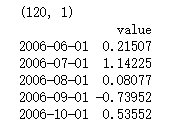# Create a training sample and testing sample before analyzing the series

n_train=int(0.95*n_sample)+1
n_forecast=n_sample-n_train
#ts_df
ts_train = ts_df.iloc[:n_train]['value']
ts_test = ts_df.iloc[n_train:]['value']
print(ts_train.shape)
print(ts_test.shape)
print("Training Series:", "\n", ts_train.tail(), "\n")
print("Testing Series:", "\n", ts_test.head())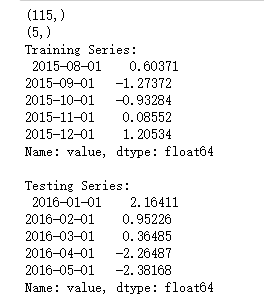def tsplot(y, lags=None, title='', figsize=(14, 8)):

fig = plt.figure(figsize=figsize)
layout = (2, 2)
ts_ax   = plt.subplot2grid(layout, (0, 0))
hist_ax = plt.subplot2grid(layout, (0, 1))
acf_ax  = plt.subplot2grid(layout, (1, 0))
pacf_ax = plt.subplot2grid(layout, (1, 1))

y.plot(ax=ts_ax) # 折线图
ts_ax.set_title(title)
y.plot(ax=hist_ax, kind='hist', bins=25) #直方图
hist_ax.set_title('Histogram')
smt.graphics.plot_acf(y, lags=lags, ax=acf_ax) # ACF自相关系数
smt.graphics.plot_pacf(y, lags=lags, ax=pacf_ax) # 偏自相关系数
[ax.set_xlim(0) for ax in [acf_ax, pacf_ax]]
sns.despine()
fig.tight_layout()
return ts_ax, acf_ax, pacf_ax

tsplot(ts_train, title='A Given Training Series', lags=20);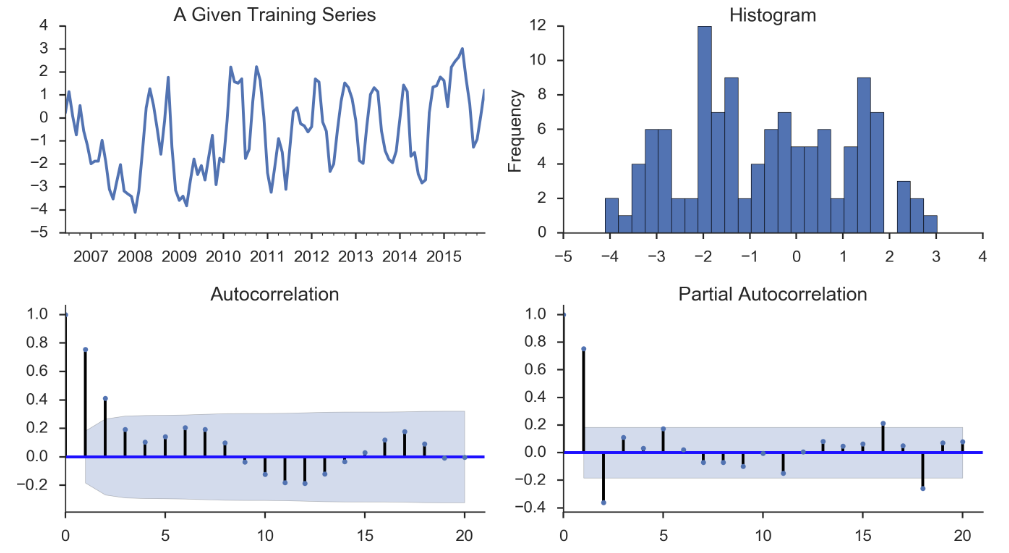# 此处运用BIC（贝叶斯信息准则）进行模型参数选择
# 另外还可以利用AIC（赤池信息准则），视具体情况而定
import itertools

p_min = 0
d_min = 0
q_min = 0
p_max = 4
d_max = 0
q_max = 4

# Initialize a DataFrame to store the results
results_bic = pd.DataFrame(index=['AR{}'.format(i) for i in range(p_min,p_max+1)],
columns=['MA{}'.format(i) for i in range(q_min,q_max+1)])

for p,d,q in itertools.product(range(p_min,p_max+1),
range(d_min,d_max+1),
range(q_min,q_max+1)):
if p==0 and d==0 and q==0:
results_bic.loc['AR{}'.format(p), 'MA{}'.format(q)] = np.nan
continue

try:
model = sm.tsa.SARIMAX(ts_train, order=(p, d, q),
#enforce_stationarity=False,
#enforce_invertibility=False,
)
results = model.fit() ##下面可以显示具体的参数结果表
## print(model_results.summary())
## print(model_results.summary().tables)
# http://www.statsmodels.org/stable/tsa.html
# print("results.bic",results.bic)
# print("results.aic",results.aic)

results_bic.loc['AR{}'.format(p), 'MA{}'.format(q)] = results.bic
except:
continue
results_bic = results_bic[results_bic.columns].astype(float)

results_bic如下所示：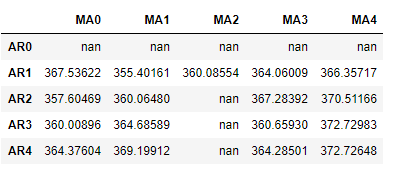为了便于观察，下面对上表进行可视化：、

fig, ax = plt.subplots(figsize=(10, 8))
ax = sns.heatmap(results_bic,
ax=ax,
annot=True,
fmt='.2f',
);
ax.set_title('BIC');
//annot
//annotate的缩写，annot默认为False，当annot为True时，在heatmap中每个方格写入数据
//annot_kws，当annot为True时，可设置各个参数，包括大小，颜色，加粗，斜体字等
# annot_kws={'size':9,'weight':'bold', 'color':'blue'}
#具体查看：https://blog.csdn.net/m0_38103546/article/details/79935671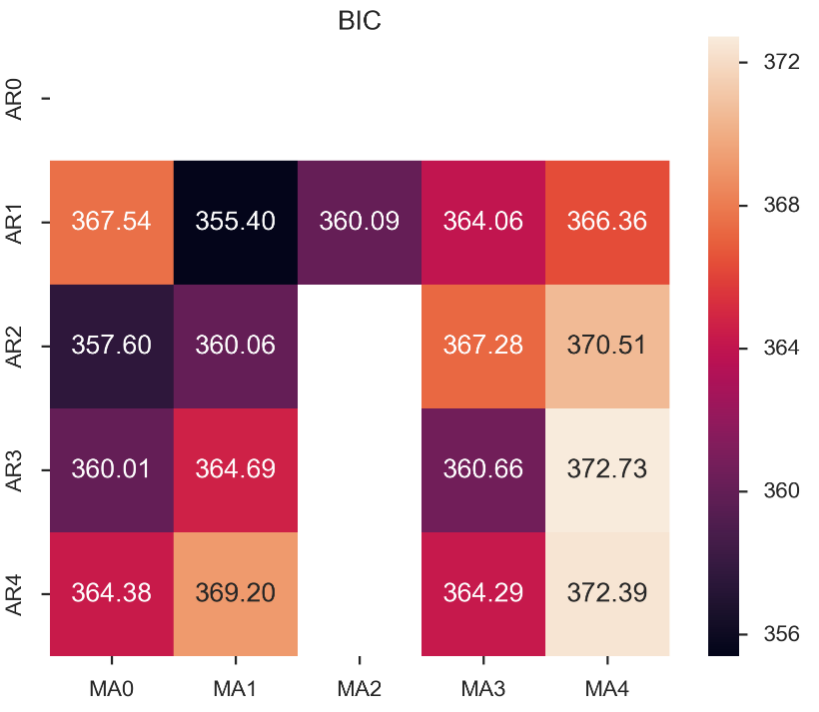# Alternative model selection method, limited to only searching AR and MA parameters

train_results = sm.tsa.arma_order_select_ic(ts_train, ic=['aic', 'bic'], trend='nc', max_ar=4, max_ma=4)

print('AIC', train_results.aic_min_order)
print('BIC', train_results.bic_min_order)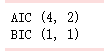注：

SARIMA总代码如下：


"""
https://www.howtoing.com/a-guide-to-time-series-forecasting-with-arima-in-python-3/
http://www.statsmodels.org/stable/datasets/index.html
"""

import warnings
import itertools
import pandas as pd
import numpy as np
import statsmodels.api as sm
import matplotlib.pyplot as plt
plt.style.use('fivethirtyeight')

'''
Most sm.datasets hold convenient representations of the data in the attributes endog and exog.
If the dataset does not have a clear interpretation of what should be an endog and exog,
then you can always access the data or raw_data attributes.
https://docs.scipy.org/doc/numpy/reference/generated/numpy.recarray.html
http://pandas.pydata.org/pandas-docs/stable/generated/pandas.DataFrame.resample.html
# resample('MS') groups the data in buckets by start of the month,
# after that we got only one value for each month that is the mean of the month
# fillna() fills NA/NaN values with specified method
# 'bfill' method use Next valid observation to fill gap
# If the value for June is NaN while that for July is not, we adopt the same value
# as in July for that in June
'''

y = data.data  # DataFrame with attributes y.columns & y.index (DatetimeIndex)
print(y)
names = data.names  # tuple
raw = data.raw_data  # float64 np.recarray

y = y['co2'].resample('MS').mean()
print(y)

y = y.fillna(y.bfill()) # y = y.fillna(method='bfill')
print(y)

y.plot(figsize=(15,6))
plt.show()

'''
2-ARIMA Parameter Seletion
ARIMA(p,d,q)(P,D,Q)s
non-seasonal parameters: p,d,q
seasonal parameters: P,D,Q
s: period of time series, s=12 for annual period
Grid Search to find the best combination of parameters
Use AIC value to judge models, choose the parameter combination whose
AIC value is the smallest
https://docs.python.org/3/library/itertools.html
http://www.statsmodels.org/stable/generated/statsmodels.tsa.statespace.sarimax.SARIMAX.html
'''

# Define the p, d and q parameters to take any value between 0 and 2
p = d = q = range(0, 2)

# Generate all different combinations of p, q and q triplets
pdq = list(itertools.product(p, d, q))

# Generate all different combinations of seasonal p, q and q triplets
seasonal_pdq = [(x, x, x, 12) for x in pdq]

print('Examples of parameter combinations for Seasonal ARIMA...')
print('SARIMAX: {} x {}'.format(pdq, seasonal_pdq))
print('SARIMAX: {} x {}'.format(pdq, seasonal_pdq))
print('SARIMAX: {} x {}'.format(pdq, seasonal_pdq))
print('SARIMAX: {} x {}'.format(pdq, seasonal_pdq))

warnings.filterwarnings("ignore") # specify to ignore warning messages

for param in pdq:
for param_seasonal in seasonal_pdq:
try:
mod = sm.tsa.statespace.SARIMAX(y,
order=param,
seasonal_order=param_seasonal,
enforce_stationarity=False,
enforce_invertibility=False)

results = mod.fit()

print('ARIMA{}x{}12 - AIC:{}'.format(param, param_seasonal, results.aic))
except:
continue

'''
3-Optimal Model Analysis
Use the best parameter combination to construct ARIMA model
Here we use ARIMA(1,1,1)(1,1,1)12
the output coef represents the importance of each feature
mod.fit() returnType: MLEResults
http://www.statsmodels.org/stable/generated/statsmodels.tsa.statespace.mlemodel.MLEResults.html#statsmodels.tsa.statespace.mlemodel.MLEResults
Use plot_diagnostics() to check if parameters are against the model hypothesis
model residuals must not be correlated
'''

mod = sm.tsa.statespace.SARIMAX(y,
order=(1, 1, 1),
seasonal_order=(1, 1, 1, 12),
enforce_stationarity=False,
enforce_invertibility=False)

results = mod.fit()
print(results.summary().tables)

results.plot_diagnostics(figsize=(15, 12))
plt.show()

'''
4-Make Predictions
get_prediction(..., dynamic=False)
Prediction of each point will use all historic observations prior to it
http://www.statsmodels.org/stable/generated/statsmodels.tsa.statespace.mlemodel.MLEResults.get_prediction.html#statsmodels.regression.recursive_ls.MLEResults.get_prediction
http://pandas.pydata.org/pandas-docs/stable/generated/pandas.Series.plot.html
https://matplotlib.org/api/_as_gen/matplotlib.axes.Axes.fill_between.html
'''

pred = results.get_prediction(start=pd.to_datetime('1998-01-01'), dynamic=False)
pred_ci = pred.conf_int() # return the confidence interval of fitted parameters

# plot real values and predicted values
# pred.predicted_mean is a pandas series
ax = y['1990':].plot(label='observed')  # ax is a matplotlib.axes.Axes

# fill_between(x,y,z) fills the area between two horizontal curves defined by (x,y)
# and (x,z). And alpha refers to the alpha transparencies
ax.fill_between(pred_ci.index,
pred_ci.iloc[:, 0],
pred_ci.iloc[:, 1], color='k', alpha=.2)

ax.set_xlabel('Date')
ax.set_ylabel('CO2 Levels')
plt.legend()

plt.show()

# Evaluation of model
y_forecasted = pred.predicted_mean
y_truth = y['1998-01-01':]

# Compute the mean square error
mse = ((y_forecasted - y_truth) ** 2).mean()
print('The Mean Squared Error of our forecasts is {}'.format(round(mse, 2)))

'''
5-Dynamic Prediction
get_prediction(..., dynamic=True)
Prediction of each point will use all historic observations prior to 'start' and
all predicted values prior to the point to predict
'''

pred_dynamic = results.get_prediction(start=pd.to_datetime('1998-01-01'), dynamic=True, full_results=True)
pred_dynamic_ci = pred_dynamic.conf_int()

ax = y['1990':].plot(label='observed', figsize=(20, 15))
pred_dynamic.predicted_mean.plot(label='Dynamic Forecast', ax=ax)

ax.fill_between(pred_dynamic_ci.index,
pred_dynamic_ci.iloc[:, 0],
pred_dynamic_ci.iloc[:, 1], color='k', alpha=.25)

ax.fill_betweenx(ax.get_ylim(), pd.to_datetime('1998-01-01'), y.index[-1],
alpha=.1, zorder=-1)

ax.set_xlabel('Date')
ax.set_ylabel('CO2 Levels')

plt.legend()
plt.show()

# Extract the predicted and true values of our time-series
y_forecasted = pred_dynamic.predicted_mean
y_truth = y['1998-01-01':]

# Compute the mean square error
mse = ((y_forecasted - y_truth) ** 2).mean()
print('The Mean Squared Error of our forecasts is {}'.format(round(mse, 2)))

'''
6-Visualize Prediction
In-sample forecast: forecasting for an observation that was part of the data sample;
Out-of-sample forecast: forecasting for an observation that was not part of the data sample.
'''

# Get forecast 500 steps ahead in future
# 'steps': If an integer, the number of steps to forecast from the end of the sample.
pred_uc = results.get_forecast(steps=500)  # retun out-of-sample forecast

# Get confidence intervals of forecasts
pred_ci = pred_uc.conf_int()

ax = y.plot(label='observed', figsize=(20, 15))
pred_uc.predicted_mean.plot(ax=ax, label='Forecast')
ax.fill_between(pred_ci.index,
pred_ci.iloc[:, 0],
pred_ci.iloc[:, 1], color='k', alpha=.25)
ax.set_xlabel('Date')
ax.set_ylabel('CO2 Levels')

plt.legend()
plt.show()



展开全文• 混沌时间序列分析与预测的常用方法。有助于整体把握混沌方法
• 该存储库实现了时间序列预测的常用方法，尤其是TensorFlow2中深度学习方法。 如果您有更好主意，欢迎您贡献力量，只需创建PR。 如有任何疑问，请随时提出问题。 正在进行项目，我将继续进行改进，因此您可能...
• 我们将首先介绍和讨论自相关，平稳性和季节性的概念，并继续应用最常用的时间序列预测方法之一，称为ARIMA。 简介：  时间序列提供了预测未来价值的机会。 基于以前的价值观，可以使用时间序列来预测经济，天气...
引言：

在本文章中，我们将提供可靠的时间序列预测。我们将首先介绍和讨论自相关，平稳性和季节性的概念，并继续应用最常用的时间序列预测方法之一，称为ARIMA。

简介：

时间序列提供了预测未来价值的机会。 基于以前的价值观，可以使用时间序列来预测经济，天气和能力规划的趋势，其中仅举几例。 时间序列数据的具体属性意味着通常需要专门的统计方法。

在本教程中，我们将针对时间序列产生可靠的预测。 我们将首先介绍和讨论自相关，平稳性和季节性的概念，并继续应用最常用的时间序列预测方法之一，称为ARIMA。

用于建模和预测时间序列未来点的Python中的一种方法被称为SARIMAX ，其代表具有eXogenous回归的季节性自动反馈集成移动平均值 。 在这里，我们将主要关注ARIMA组件，该组件用于适应时间序列数据，以更好地了解和预测时间序列中的未来点。

1 实验条件

本将介绍如何在本地桌面或远程服务器上进行时间序列分析。 使用大型数据集可能是内存密集型的，所以在这两种情况下，计算机将至少需要2GB的内存来执行本指南中的一些计算。

我使用的IDE: PyCharm ，实验Python版本：3.6

实验所用的库： pandas(操作数据)    statsmodels(统计分析库)    matplotlib(可视化)   itertools(迭代工具)   warnings(设置警告)

引入包：

import pandas as pd
import statsmodels.api as sm
import matplotlib.pyplot as plt
import matplotlib as mpl
import itertools
import warnings

2 数据集：

我们将使用一个名为“来自美国夏威夷Mauna Loa天文台的连续空气样本的大气二氧化碳”的数据集，该数据集从1958年3月至2001年12月期间收集了二氧化碳样本。这些数据可以通过statsmodels.api 引进来；

#引入数据
#对引入的数据进行变形
index = pd.DatetimeIndex(start=data.data['date'].decode('utf-8'),
periods=len(data.data),freq='W-SAT')
co2=pd.DataFrame(data.data['co2'], index=index, columns=['co2'])
print(co2.index)#检查co2的索引

运行结果：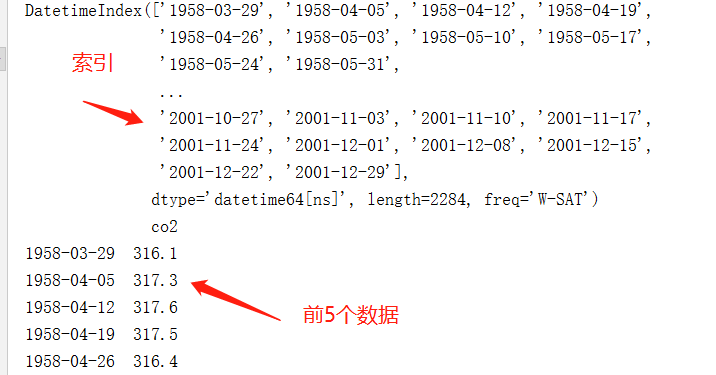我们稍后再进行一些数据处理。从上面的的结果可以看出，每周数据可能很棘手，因为它是一个很短的时间，所以让我们使用每月平均值。 我们将使用resample函数进行转换。 另外当数据量比较大的时候，容易存在缺失值，我们需要先检测是否存在缺失值，如果缺失值过多，则说明数据不可用，如果数据缺失的不太多，可以用一些方法进行拟合补全。为了简单起见，这里 我们使用fillna()函数来确保我们的时间序列中没有缺少值。当然可以自己训练模型根据预测结果，进行自动补全。

y=co2["co2"].resample("MS").mean()#获得每个月的平均值
print(y.isnull().sum)#5个 检测空白值
#处理数据中的缺失项
y=y.fillna(y.bfill())#填充缺失值

经过上部，我们已经补全了所有的空白值。

3 初始数据可视化：

我们可以先看看原始的数据，可视化的展示：

plt.figure(figsize=(15,6))
plt.title("原始数据",loc="center",fontsize=20)
plt.plot(y)

实验结果：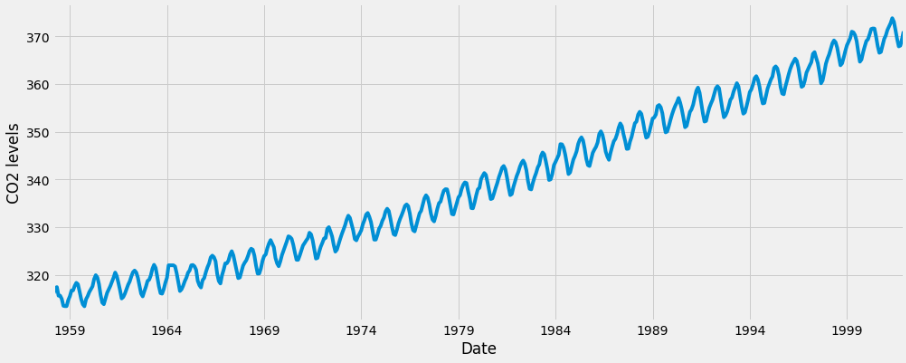由实验结果可以看出：当我们绘制数据时，会出现一些可区分的模式。 时间序列具有明显的季节性格局，总体呈上升趋势。

现在我们已经转换和探索了原始的数据，接下来我们继续使用ARIMA进行时间序列预测。

4 ARIMA时间序列模型

时间序列预测中最常用的方法之一就是被称为ARIMA模型，它代表了A utoreg R essive综合M oving A版本 。 ARIMA是可以适应时间序列数据的模型，以便更好地了解或预测系列中的未来点。

有三个不同的整数（ p ， d ， q ）用于参数化ARIMA模型。 因此，ARIMA模型用符号ARIMA(p, d, q) 。 这三个参数共计数据集中的季节性，趋势和噪音：

p是模型的自回归部分。 它允许我们将过去价值观的影响纳入我们的模型。 直观地说，这将是类似的，表示如果过去3天已经变暖，明天可能会变暖。
d是模型的集成部分。 这包括模型中包含差异量（即从当前值减去的过去时间点的数量）以适用于时间序列的术语。 直观地说，这将类似于说如果过去三天的温度差异非常小，明天可能会有相同的温度。
q是模型的移动平均部分。 这允许我们将模型的误差设置为过去以前时间点观察到的误差值的线性组合。
在处理季节性影响时，我们利用季节性 ARIMA，表示为ARIMA(p,d,q)(P,D,Q)s 。 这里， (p, d, q)是上述非季节性参数，而(P, D, Q)遵循相同的定义，但适用于时间序列的季节分量。 术语s是时间序列的周期（季度为4 ，年度为12 ，等等）。

由于所涉及的多个调整参数，季节性ARIMA方法可能会令人望而生畏。所以这里我们采用年度的预测。 在下一节中，我们将介绍如何自动化识别季节性ARIMA时间序列模型的最优参数集的过程。

5  ARIMA时间序列模型的参数选择

当考虑使用季节性ARIMA模型拟合时间序列数据时，我们的第一个目标是找到优化感兴趣度量的ARIMA(p,d,q)(P,D,Q)s的值。 实现这一目标有许多指导方针和最佳实践，但ARIMA模型的正确参数化可能是一个需要领域专长和时间的艰苦的手工过程。

这里我们使用“网格搜索”来迭代地探索参数的不同组合。 对于参数的每个组合，我们使用statsmodels模块的SARIMAX()函数拟合一个新的季节性ARIMA模型，并评估其整体质量。 一旦我们探索了参数的整个范围，我们的最佳参数集将是我们感兴趣的标准产生最佳性能的参数。 我们开始生成我们希望评估的各种参数组合：

#找合适的p d q
#初始化 p d q
p=d=q=range(0,2)
print("p=",p,"d=",d,"q=",q)
#产生不同的pdq元组,得到 p d q 全排列
pdq=list(itertools.product(p,d,q))
print("pdq:\n",pdq)
seasonal_pdq=[(x,x,x,12) for x in pdq]
print('SQRIMAX:{} x {}'.format(pdq,seasonal_pdq))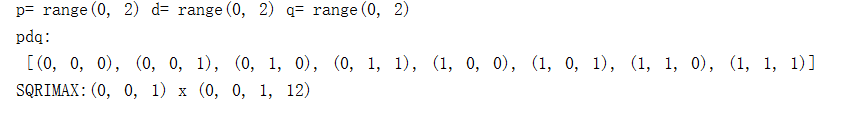我们现在可以使用上面定义的参数三元组来自动化不同组合对ARIMA模型进行培训和评估的过程。 在统计和机器学习中，这个过程被称为模型选择的网格搜索（或超参数优化）。

在评估和比较配备不同参数的统计模型时，可以根据数据的适合性或准确预测未来数据点的能力，对每个参数进行排序。 我们将使用AIC （Akaike信息标准）值，该值通过使用statsmodels安装的ARIMA型号方便地返回。 AIC衡量模型如何适应数据，同时考虑到模型的整体复杂性。 在使用大量功能的情况下，适合数据的模型将被赋予比使用较少特征以获得相同的适合度的模型更大的AIC得分。 因此，我们有兴趣找到产生最低AIC值的模型。

下面的代码块通过参数的组合来迭代，并使用SARIMAX函数来适应相应的季节性ARIMA模型。 这里， order参数指定(p, d, q)参数，而seasonal_order参数指定季节性ARIMA模型的(P, D, Q, S)季节分量。 在安装每个SARIMAX()模型后，代码打印出其各自的AIC得分。

for param in pdq:
for param_seasonal in seasonal_pdq:
try:
mod = sm.tsa.statespace.SARIMAX(y,
order=param,
seasonal_order=param_seasonal,
enforce_stationarity=False,
enforce_invertibility=False)

results = mod.fit()

print('ARIMA{}x{}12 - AIC:{}'.format(param, param_seasonal, results.aic))
except:
continue

运行结果：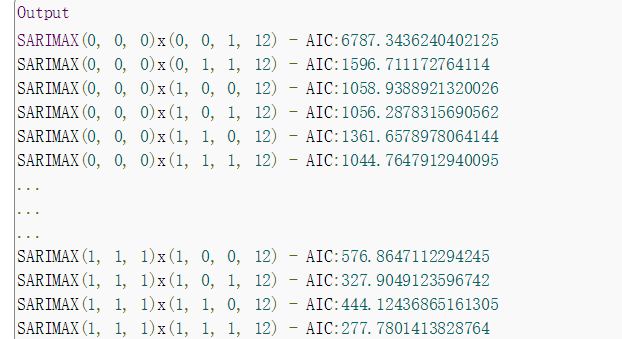【注：实验结果会有很多的数据，这里只截取了AIC的结果，且需要执行一会儿】

我们的代码的输出表明， SARIMAX(1, 1, 1)x(1, 1, 1, 12)产生最低的AIC值为277.78。 因此，我们认为这是我们考虑过的所有模型中的最佳选择。

6 配置ARIMA时间序列模型

利用以上的估计，使该模型为 (p,d,q)=(1,1,1)

mod = sm.tsa.statespace.SARIMAX(y,
order=(1, 1, 1),
seasonal_order=(1, 1, 1, 12),
enforce_stationarity=False,
enforce_invertibility=False)

results = mod.fit()

print(results.summary().tables)

结果：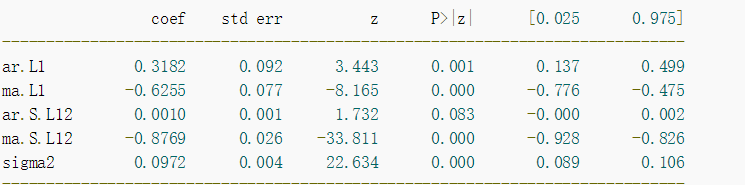由SARIMAX的输出产生的SARIMAX返回大量的信息，但是我们将注意力集中在系数表上。 coef列显示每个特征的重量（即重要性）以及每个特征如何影响时间序列。 P>|z| 列通知我们每个特征重量的意义。 这里，每个重量的p值都低于或接近0.05 ，所以在我们的模型中保留所有权重是合理的。

在适合季节性ARIMA模型（以及任何其他模型）的情况下，运行模型诊断是非常重要的，以确保没有违反模型的假设。 plot_diagnostics对象允许我们快速生成模型诊断并调查任何异常行为。

results.plot_diagnostics(figsize=(15, 12))
plt.show()

运行结果：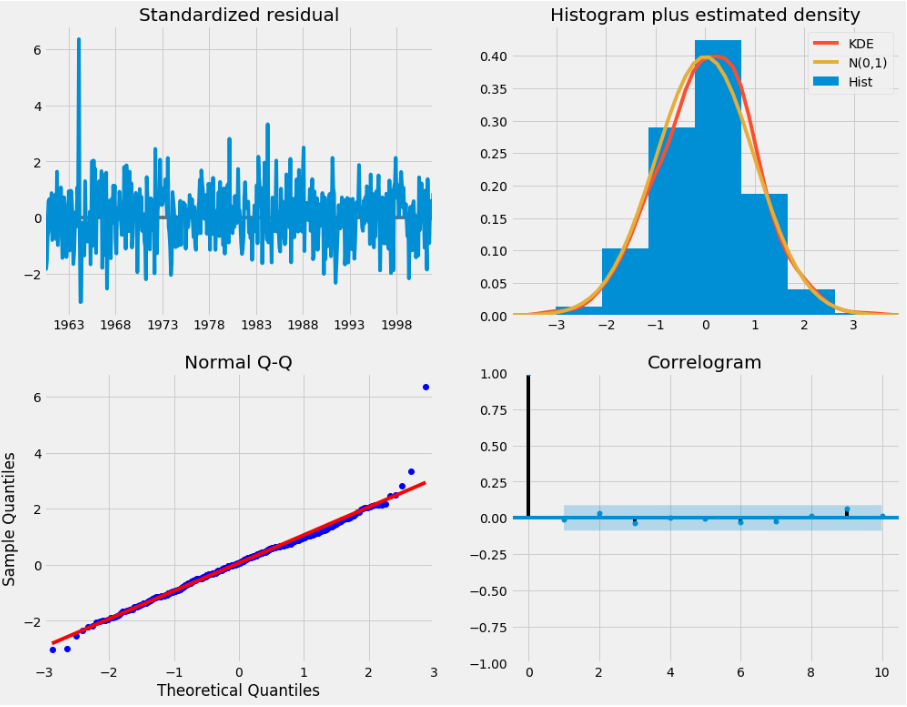我们的主要关切是确保我们的模型的残差是不相关的，并且平均分布为零。 如果季节性ARIMA模型不能满足这些特性，这是一个很好的迹象，可以进一步改善。

在这种情况下，我们的模型诊断表明，模型残差正常分布如下：

在右上图中，我们看到红色KDE线与N(0,1)行（其中N(0,1) ）是正态分布的标准符号，平均值0 ，标准偏差为1 ） 。 这是残留物正常分布的良好指示。

左下角的qq图显示，残差（蓝点）的有序分布遵循采用N(0, 1)的标准正态分布采样的线性趋势。 同样，这是残留物正常分布的强烈指示。

随着时间的推移（左上图）的残差不会显示任何明显的季节性，似乎是白噪声。 这通过右下角的自相关（即相关图）来证实，这表明时间序列残差与其本身的滞后版本具有低相关性。

这些观察结果使我们得出结论，我们的模型产生了令人满意的合适性，可以帮助我们了解我们的时间序列数据和预测未来价值。

虽然我们有一个令人满意的结果，我们的季节性ARIMA模型的一些参数可以改变，以改善我们的模型拟合。 例如，我们的网格搜索只考虑了一组受限制的参数组合，所以如果我们拓宽网格搜索，我们可能会找到更好的模型。

7 验证预测

我们已经获得了我们时间序列的模型，现在可以用来产生预测。 我们首先将预测值与时间序列的实际值进行比较，这将有助于我们了解我们的预测的准确性。 get_prediction()和conf_int()属性允许我们获得时间序列预测的值和相关的置信区间。

# #进行验证预测
pred=results.get_prediction(start=pd.to_datetime('1998-01-01'),dynamic=False)
pred_ci=pred.conf_int()
print("pred ci:\n",pred_ci)#获得的是一个预测范围，置信区间
print("pred:\n",pred)#为一个预测对象
print("pred mean:\n",pred.predicted_mean)#为预测的平均值

上述规定需要从1998年1月开始进行预测。

dynamic=False参数确保我们产生一步前进的预测，这意味着每个点的预测都将使用到此为止的完整历史生成。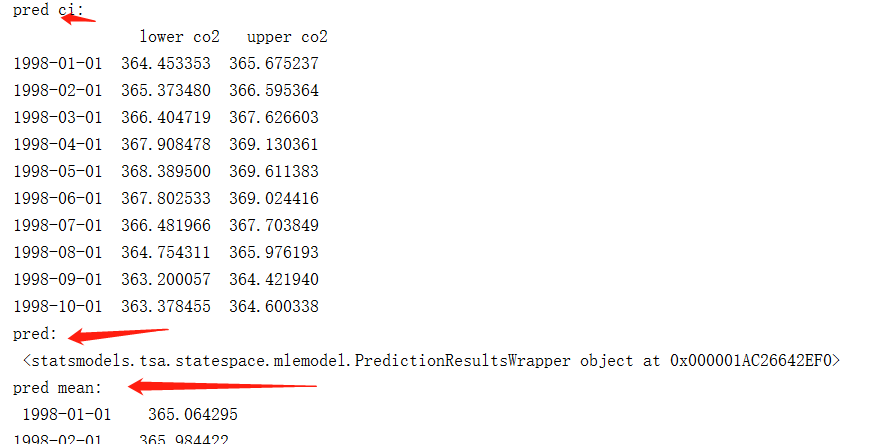我们可以绘制二氧化碳时间序列的实际值和预测值，以评估我们做得如何。 注意我们如何在时间序列的末尾放大日期索引。

#进行绘制预测图像
ax=y['1990':].plot(label="observed")
pred.predicted_mean.plot(ax=ax,label="static ForCast",alpha=.7,color='red',linewidth=5)
#在某个范围内进行填充
ax.fill_between(pred_ci.index,
pred_ci.iloc[:, 0],
pred_ci.iloc[:, 1], color='k', alpha=.2)
ax.set_xlabel('Date')
ax.set_ylabel('CO2 Levels')
plt.legend()
plt.show()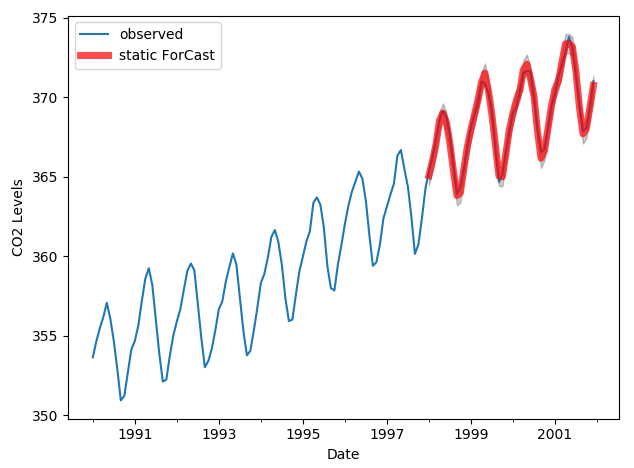总体而言，我们的预测与真实价值保持一致，呈现总体增长趋势。

量化我们的预测的准确性也是有用的。 我们将使用MSE（均方误差），它总结了我们的预测的平均误差。 对于每个预测值，我们计算其到真实值的距离并对结果求平方。 结果需要平方，以便当我们计算总体平均值时，正/负差异不会相互抵消。

# 求取 MSE（均方误差）
y_forecasted=pred.predicted_mean
y_truth=y['1998-01-01':]
mse=((y_forecasted-y_truth)**2).mean()
print("MSE:",mse)

结果：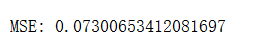我们前进一步预测的MSE值为0.07 ，这是接近0的非常低的值。0的MSE是估计器将以完美的精度预测参数的观测值，这将是一个理想的场景但通常不可能。

然而，使用动态预测可以获得更好地表达我们的真实预测能力。 在这种情况下，我们只使用时间序列中的信息到某一点，之后，使用先前预测时间点的值生成预测。

在下面的代码块中，我们指定从1998年1月起开始计算动态预测和置信区间

pred_dynamic = results.get_prediction(start=pd.to_datetime('1998-01-01'), dynamic=True, full_results=True)
pred_dynamic_ci = pred_dynamic.conf_int()

绘制时间序列的观测值和预测值，我们看到即使使用动态预测，总体预测也是准确的。 所有预测值（红线）与地面真相（蓝线）相当吻合，并且在我们预测的置信区间内。

# #使用动态预测
pred_dynamic = results.get_prediction(start=pd.to_datetime('1998-01-01'), dynamic=True, full_results=True)
pred_dynamic_ci = pred_dynamic.conf_int()
ax = y['1990':].plot(label='observed', figsize=(20, 15))
pred_dynamic.predicted_mean.plot(label='Dynamic Forecast', ax=ax)

ax.fill_between(pred_dynamic_ci.index,
pred_dynamic_ci.iloc[:, 0],
pred_dynamic_ci.iloc[:, 1], color='k', alpha=.25)

ax.fill_betweenx(ax.get_ylim(), pd.to_datetime('1998-01-01'), y.index[-1],
alpha=.1, zorder=-1)

ax.set_xlabel('Date')
ax.set_ylabel('CO2 Levels')

plt.legend()
plt.show()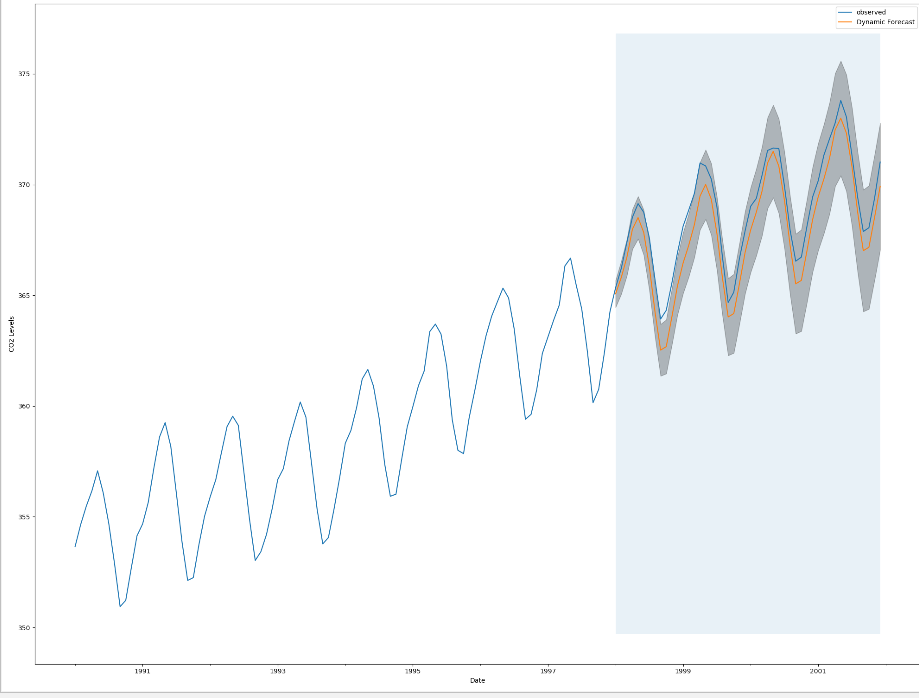我们再次通过计算MSE量化我们预测的预测性能：

# Extract the predicted and true values of our time-series
y_forecasted = pred_dynamic.predicted_mean
y_truth = y['1998-01-01':]

# Compute the mean square error
mse = ((y_forecasted - y_truth) ** 2).mean()
print('The Mean Squared Error of our forecasts is {}'.format(round(mse, 2)))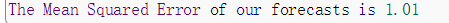从动态预测获得的预测值产生1.01的MSE。 这比前进一步略高，这是预期的，因为我们依赖于时间序列中较少的历史数据。

前进一步和动态预测都证实了这个时间序列模型是有效的。 然而，关于时间序列预测的大部分兴趣是能够及时预测未来价值观。

8 生成和可视化预测

我们将介绍如何利用季节性ARIMA时间序列模型来预测未来的价值。 我们的时间序列对象的get_forecast()属性可以计算预先指定数量的步骤的预测值。

# Get forecast 500 steps ahead in future
pred_uc = results.get_forecast(steps=200)#steps 可以代表（200/12）年左右

# Get confidence intervals of forecasts
pred_ci = pred_uc.conf_int()
plt.title("预测",fontsize=15,color="red")
ax = y.plot(label='observed', figsize=(20, 15))
pred_uc.predicted_mean.plot(ax=ax, label='Forecast')
ax.fill_between(pred_ci.index,
pred_ci.iloc[:, 0],
pred_ci.iloc[:, 1], color='k', alpha=.25)
ax.set_xlabel('Date',fontsize=15)
ax.set_ylabel('CO2 Levels',fontsize=15)

plt.legend()
plt.show()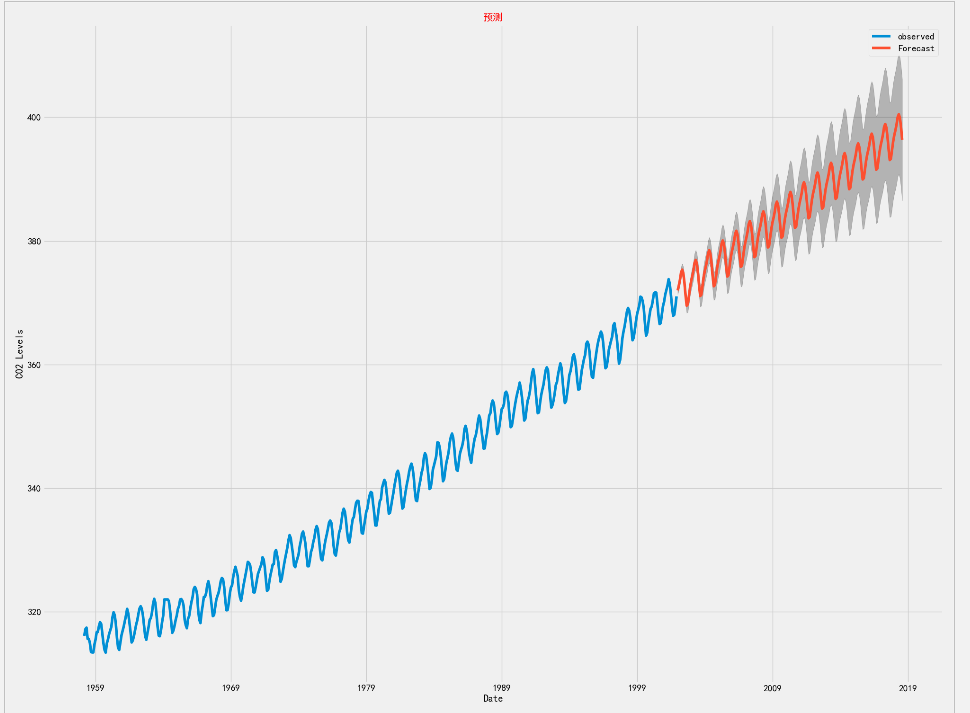现在可以使用我们生成的预测和相关的置信区间来进一步了解时间序列并预见预期。 我们的预测显示，时间序列预计将继续稳步增长。

随着我们对未来的进一步预测，我们对自己的价值观信心愈来愈自然。 这反映在我们的模型产生的置信区间，随着我们进一步走向未来，这个模型越来越大。

9 结论

在本教程中，我们描述了如何在Python中实现季节性ARIMA模型。 我们广泛使用了pandas和statsmodels图书馆，并展示了如何运行模型诊断，以及如何产生二氧化碳时间序列的预测。

这里还有一些其他可以尝试的事情：

更改动态预测的开始日期，以了解其如何影响预测的整体质量。
尝试更多的参数组合，看看是否可以提高模型的适合度。
选择不同的指标以选择最佳模型。 例如，我们使用AIC测量来找到最佳模型，但是您可以寻求优化采样均方误差。
对于更多的实践，您还可以尝试加载另一个时间序列数据集来生成您自己的预测。

【本篇文章源自前辈的实验，因为前辈描述的较为详细，这里只是做了细微的调整。原文地址：https://www.howtoing.com/a-guide-to-time-series-forecasting-with-arima-in-python-3/】
展开全文• ## 时间序列常用方法

千次阅读 2017-07-03 10:56:54
时间序列分析基本特征： ...1.时间序列分析法是根据过去变化趋势预测未来发展,它前提是假定事物过去延续到未来。 2.时间序列数据变动存在着规律性与不规律性。 时间序列每个观察值
• 我们将首先介绍和讨论自相关，平稳性和季节性的概念，并继续应用最常用的时间序列预测方法之一，称为ARIMA。 2 简介 时间序列提供了预测未来价值的机会。 基于以前的价值观，可以使用时间序列来预测经济，天气和能力...
• 该教程通过一个简单实例浅显描述了时间序列预测的常用方法。选用的方法是EXCEL插件XLMiner，简单易上手，是新手入门好帮手。
• Python的科学计算和数据挖掘相关库中，pandas和statsmodels都提供了时间序列相关分析功能，本示例使用的是statsmodels做时间序列预测应用。有关时间序列算法的选择，实际场景中最常用的是ARIMA或ARMA了，因此本示例...
• 针对特定的预测问题，只是拥有数据还不够，想要从纷繁复杂数据关系中挖掘出可用于预测的规律或模式，还得运用恰当分析方法。比如聚类分析，恰当地选择聚类算法，可以按维度将数据适当地分群，根据各类特征制订...
• ## 平稳时间序列预测

千次阅读 2017-06-24 09:43:47
所谓预测，就是要利用序列已知的样本值对序列在未来的某个时刻的取值进行估计。目前对平稳序列常用的预测方法是线性最小方差预测。线性是指预测值为观察值序列的线性函数，最小方差是指预测方差达到最小。
• 时间序列法是一种定量预测方法，亦称简单外延方法。在统计学中作为一种常用的预测手段被广泛应用。
• 在本教程中，我们将首先介绍和讨论自相关，平稳性和季节性的概念，然后继续应用最常用的时间序列预测方法之一，称为ARIMA。 Python中可用的一种用于建模和预测时间序列的未来点的方法称为SARIMAX，它表示带有季节性...python
• 自回归模型，模型参量法高分辨率谱分析方法之一，也是现代谱估计中常用的模型。如果{εt}\left\{ \varepsilon _{t}\right\}{εt​}为白噪声，服从N(0,σ2)N\left( 0,\sigma ^{2}\right)N(0,σ2)，a0,a1,...,ap(ap≠0...机器学习 tensorflow 算法
• 回声状态网络(ESN) 学习算法中可能存在解奇异问题, 在时间序列预测时易导致病态解问题, 且伴随 着具有较大幅值输出权值, 尤其是当训练样本个数小于输出权值维数时, ESN 解必为奇异. 鉴于此, 考虑使 ...
• 1.2 常用符号：时间，时间序列数据，预测值，预测误差 1.3 预测过程：定义预测目标，收集并整理数据，进行探索性分析，根据数据特点选择预测方法，进行预测分析。根据预测目标选择合适指标，计算预测精确度，对...
• 多元回归方法仍是最常用的多变量预测方法，但对经济时间序列拟合多元回归模型存在一些问题，于是人们对向量回归模型进行了大量的研究。本文着重分析了国外学者关于预测方法的选择以及非线性模型的研究动态。
• 时间序列是按照一定的时间间隔取得的一系列观测值，是同一对象在不同时间点所表现出的特征。不同于横截面数据，是同一时间点不同地点，不同事件所表现的特征，关注的维度不同。 时间序列分析是干什么？ 时间序列分析...
• 加权一阶局域预测法是目前最常用的一种混沌时间序列预测方法。基于延迟坐标相空间重构理论，提出了混沌时间序列改进的加权一阶局域预测法。仿真结果表明该方法的多步预测性能与一步预测性能明显好于加权一阶局域预测...
• 常用的模型，以下基本可以涵盖主流思想： 传统时序模型：ARIMA，Prophet，EMD 构造时序特征的统计学习方法：LR，GBDT(xgboost\lightgbm) 深度学习方法：seq2seq，wavenet，transormer 企业研究 来自工业届的研究...机器学习 深度学习 python
• 文章目录时间序列预测算法总结前言一、基于统计时序数据建模方法1.1传统时序数据建模方法1.1.1周期因子法1.1.2移动平均法1.1.3ARIMA模型1.1.3.1模型原理1.平稳性要求2.AR模型3.MA模型4.ARMA模型5.ARIMA模型1.1.3.2...机器学习 深度学习
• 根据瓦斯涌出特征,分析了目前常用瓦斯预测方法的适用条件,在假定瓦斯浓度只受时间和历史数据影响情况下,运用时间序列分析方法对工作面瓦斯浓度变化规律进行了预测,结果表明,利用时间序列分析方法预测工作面...
• 指数平滑法是在移动平均法基础上发展起来的一种时间序列分析预测法，它是通过计算指数平滑值，配合一定的时间序列预测模型对现象的未来进行预测。其原理是任一期的指数平滑值都是本期实际观察值与前一期指数平滑值的...算法
• 预测时间序列上，指数平滑法是另一类常用的方法。该方法最先由布朗提出，他认为时间序列的态势具有稳定性或规则性，所以可被合理地顺势推延；最近发生的，在某种程度上会持续到最近的未来，所以历史信息越新，其所...excel 平滑滚动 excel平滑滚动
• 混沌时间序列分析与预测工具箱 Version2.0，该工具箱包括了混沌时间序列分析与预测的常用方法,有:产生混沌时间序列(chaotic time series)等
• 也用于中短期经济发展趋势预测，所有预测方法中，指数一次指数平滑法平滑是用得最多一种。在时间序列中，我们需要基于该时间序列当前已有数据来预测其在之后走势，三次指数平滑(Triple/Three Order ...
• 　移动平均法是一种简单平滑预测技术，它基本思想是：根据时间序列资料、逐项推移，依次计算包含一定项数序时平均值，以反映长期趋势的方法。因此，当时间序列的数值由于受周期变动和随机波动影响，起伏较大.java
• 其中,大量的时间序列问题广泛分布在实现生活的许多领域中，对时间序列的分析我们也称之为趋势预测探索、更复杂的非平稳时间序列模型等。机器学习应用中，比较复杂的平稳时间序列模型、更复杂的非平稳时间序列模型等...机器学习...